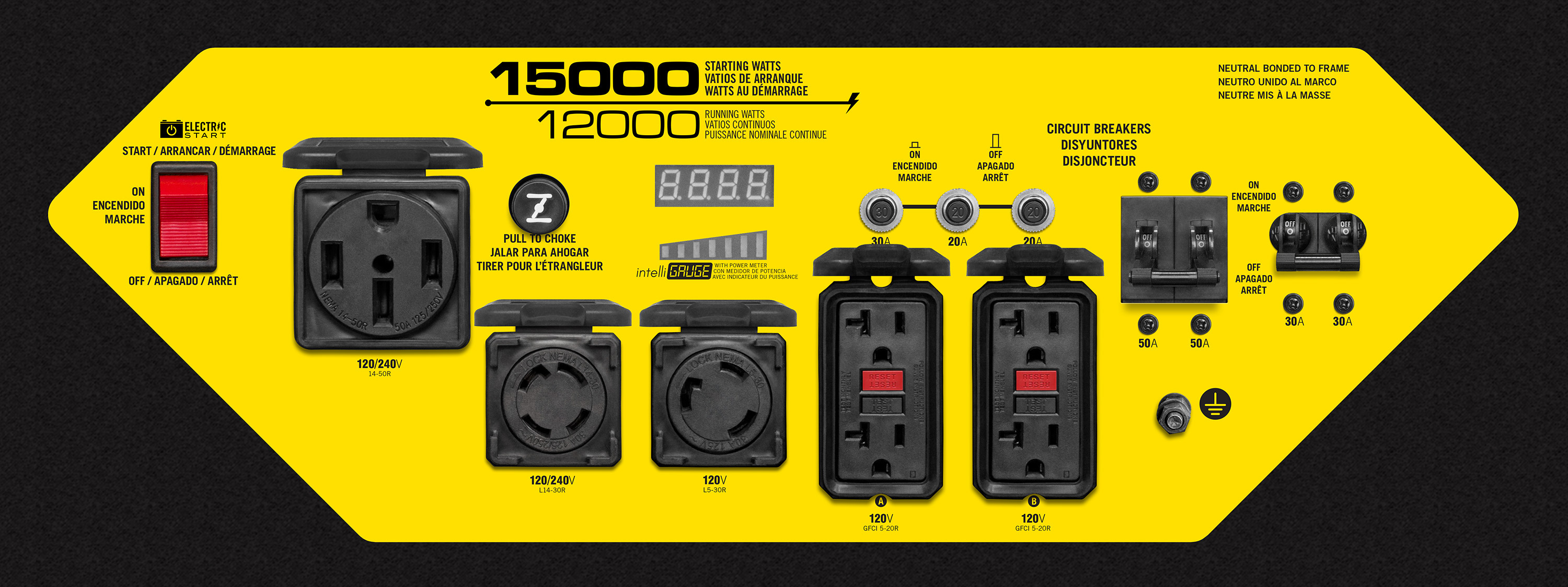# Portable Generator Intelligauge Display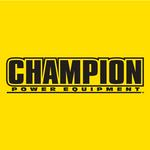Updated 1 year ago by Juan Velez

This article will show you how to read your generator's intelligauge. What the meter displays can also be found in the owner's manual. All models shown here are our current listing of portable generators.

Scroll through the article to find your generator's wattage rating or hold Ctrl + F if you're on Windows to bring up a search bar; Command + F on Mac.

1200-Watt Generators

Model: 100404, 100458, 200915
Cycles through total run time, voltage, and hertz.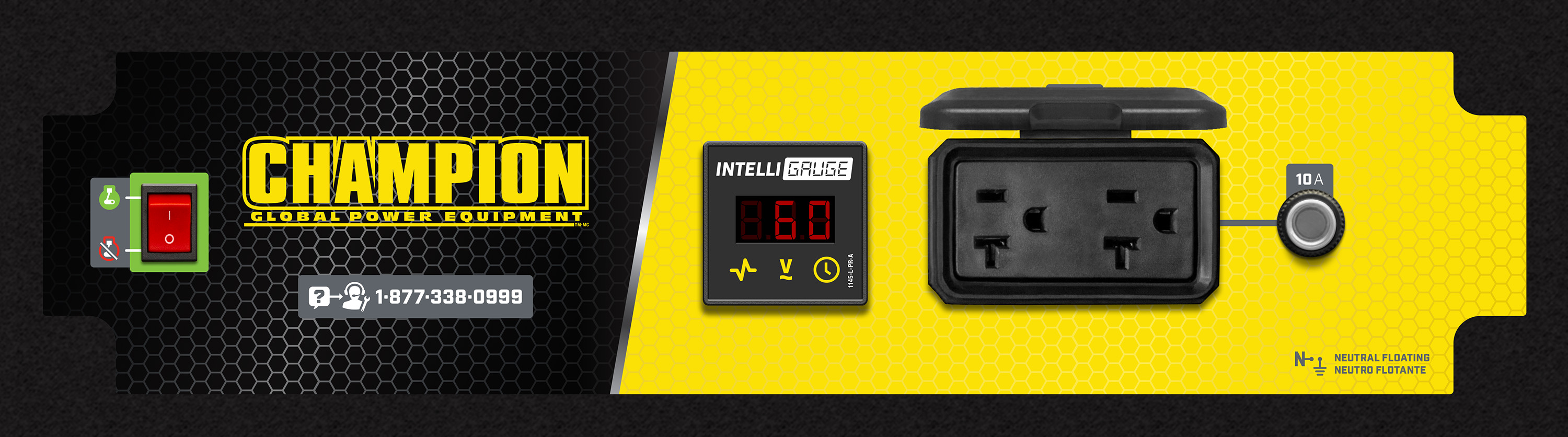2000-Watt Inverters

Model: 100402, 100745, 100306, 100705, 100414
Displays total run time (first five seconds after unit started), power output, maintenance light, low oil level indicator, receptacle status, and fuel level indicator.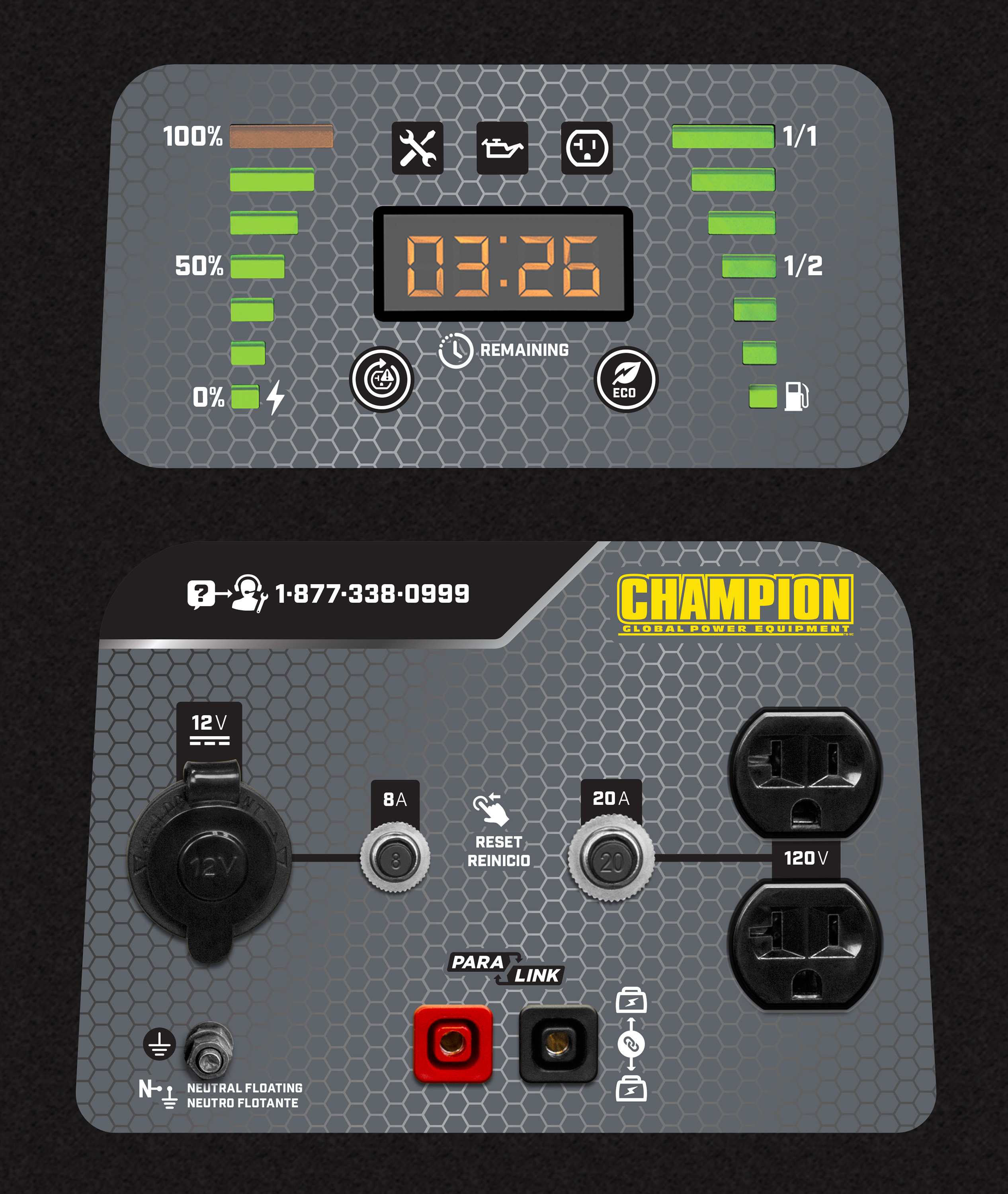2500-Watt Inverters

Model: 201153
Cycles through voltage, frequency, run time, and total run time.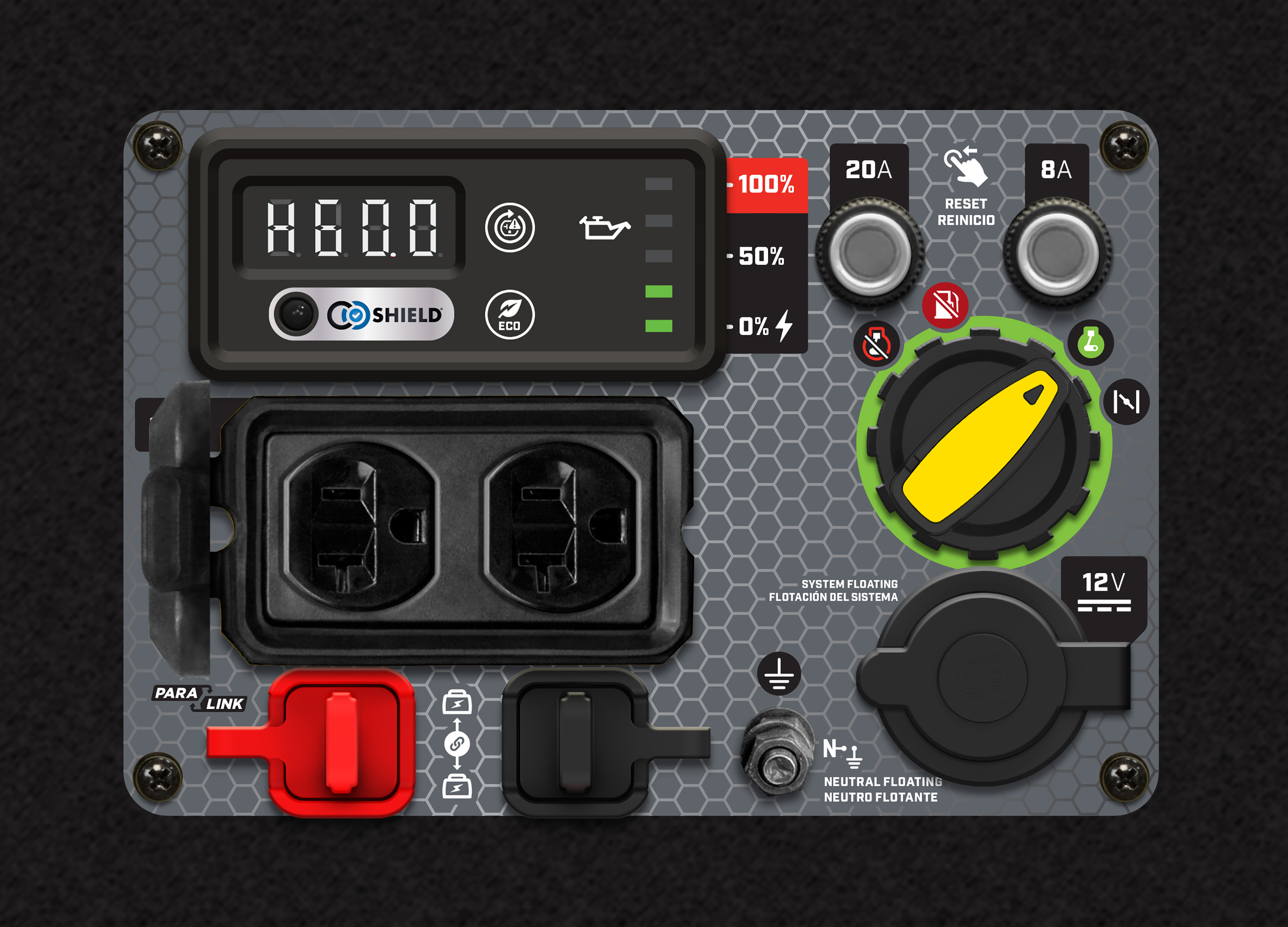3500-Watt Generators

Model: 200965, 200966, 200967
Cycles through voltage, frequency, run time, and total run time.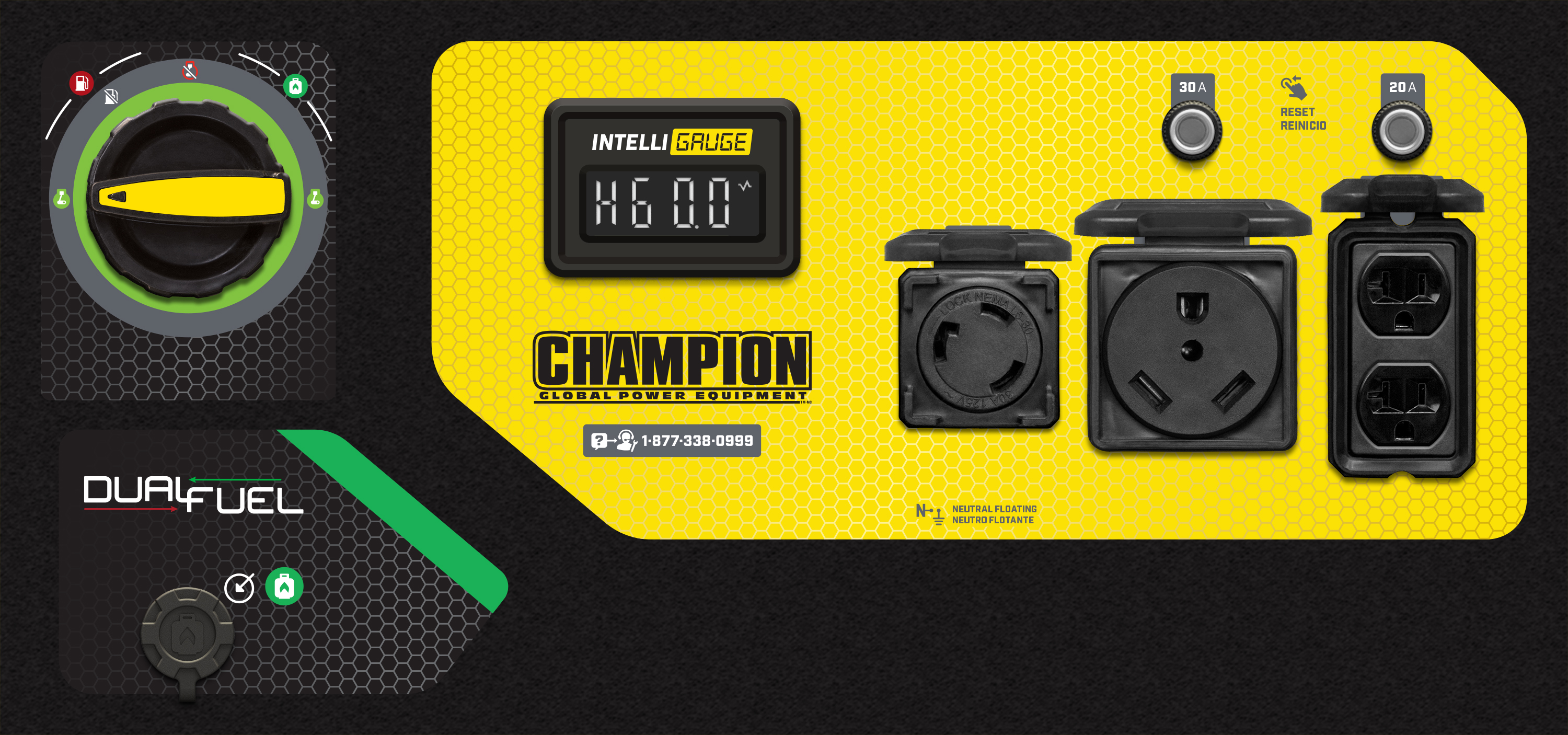Model: 100307, 100307-1
Cycles through voltage, hertz, and total run time.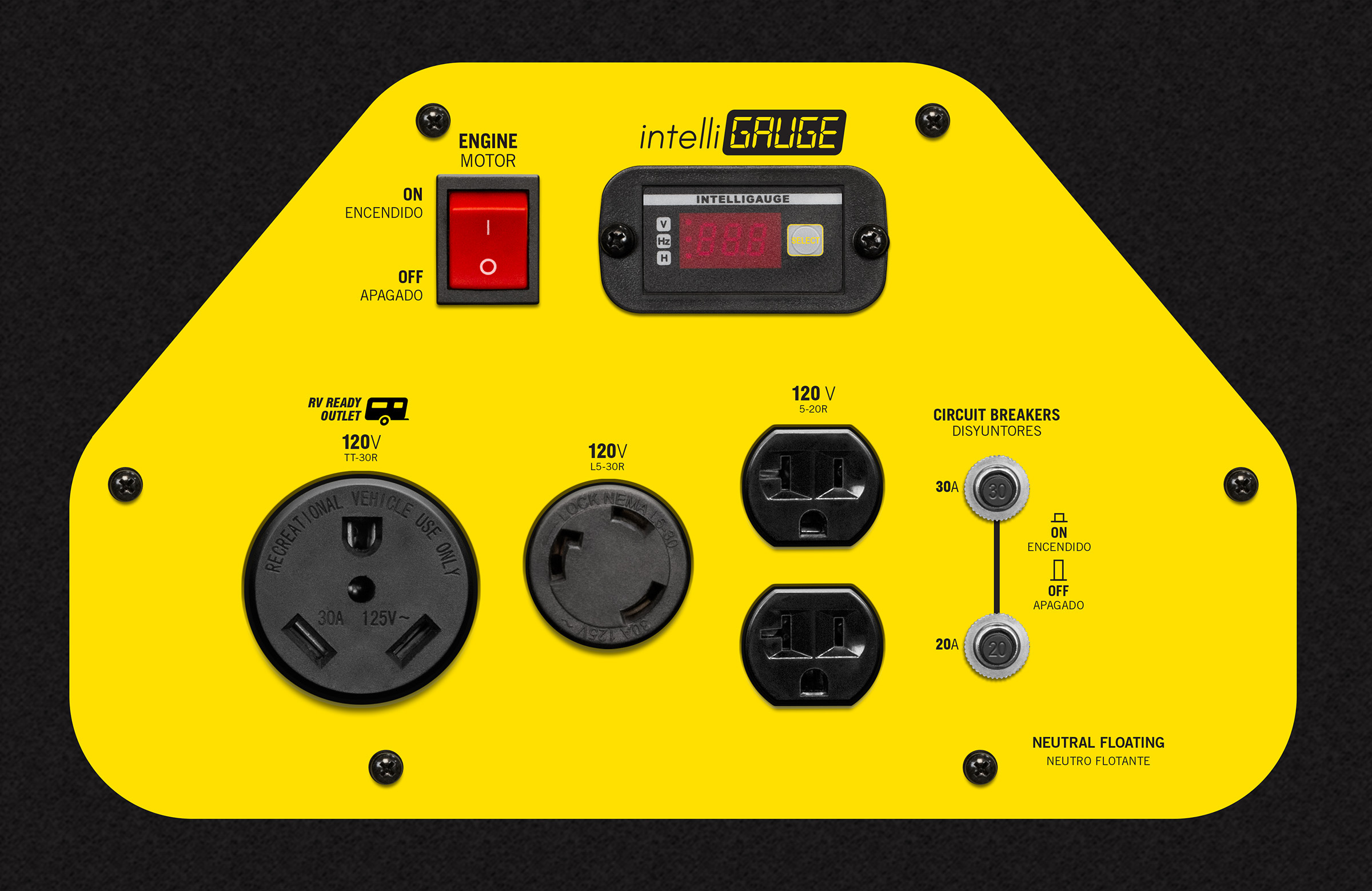Model: 100522, 100554, 100555, 100559
Cycles through voltage, frequency (hertz), run time, and total run time.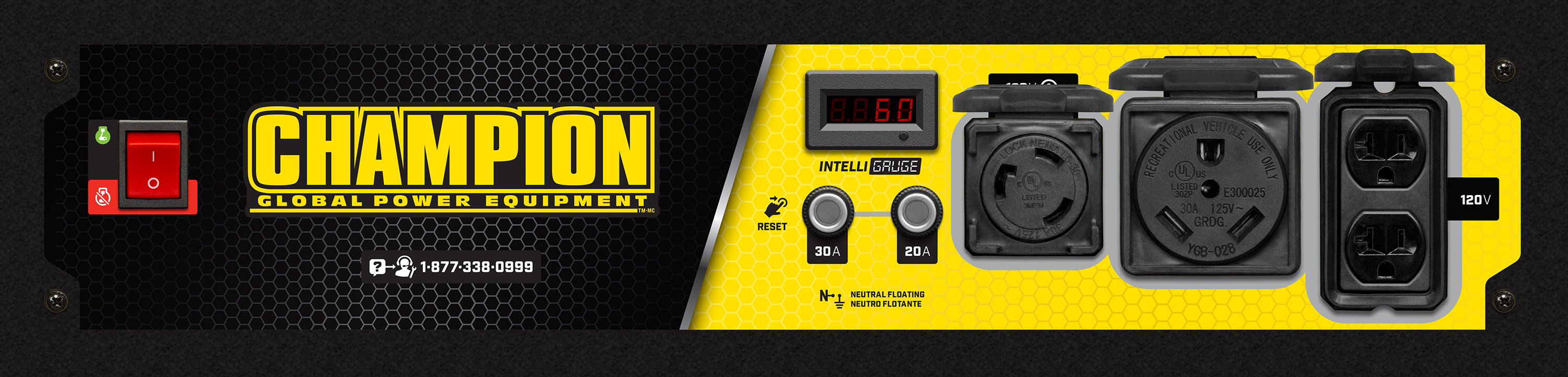Model: 200963, 200964
Cycles frequency (hertz), voltage, and total run time.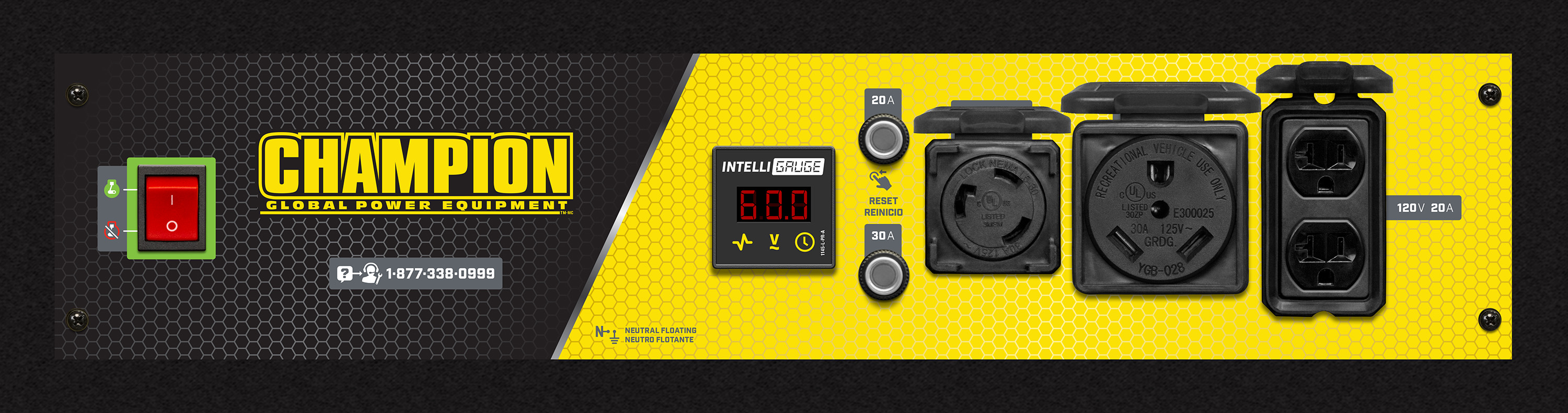3550-Watt Generators

Model: 100406, 100415, 100459, 100460, 200911, 201042
Cycles through running time, voltage, and hertz.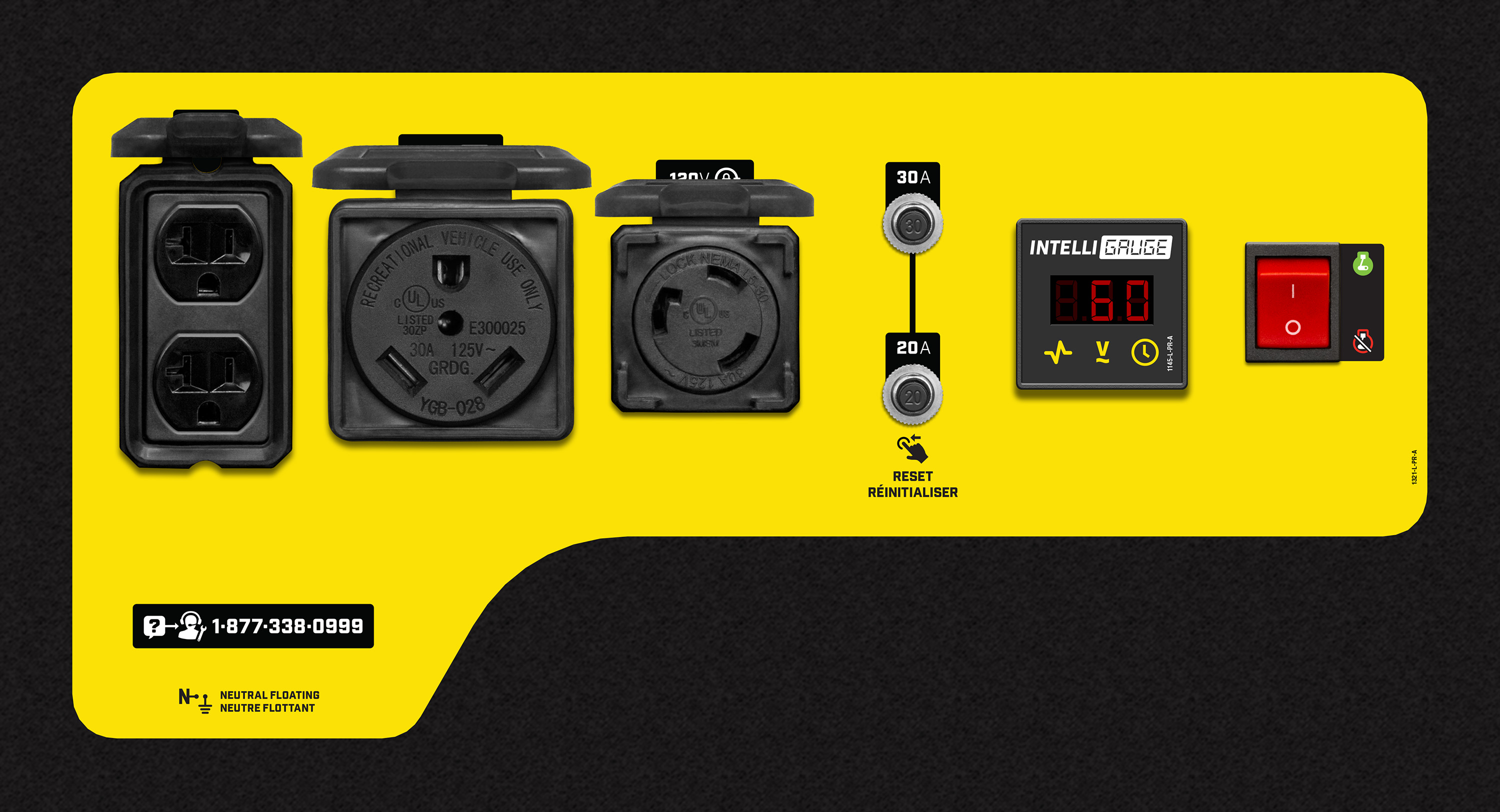3650-Watt Generators

Model: 200972, 200973
Cycles through voltage, frequency, run time, and total run time.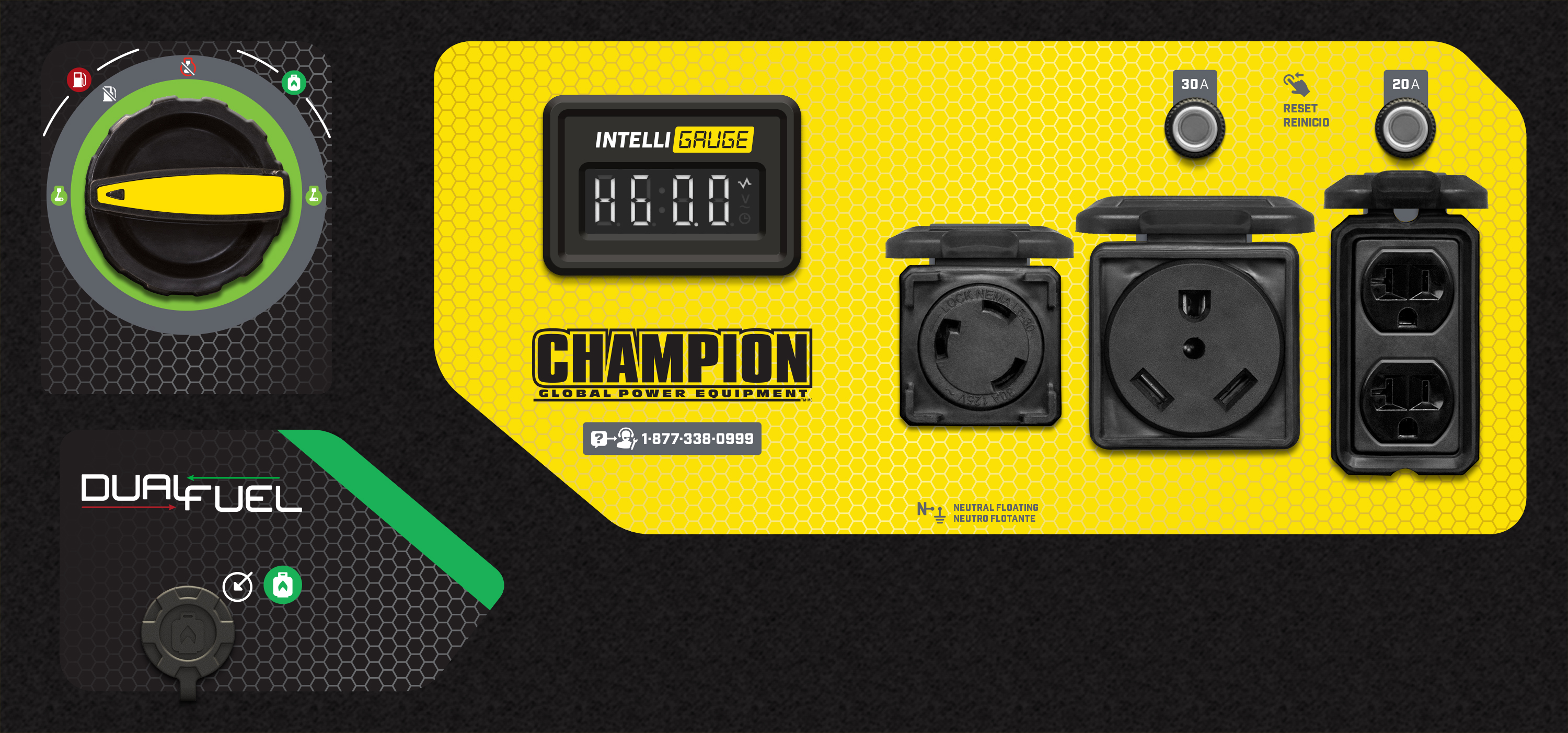Model: 200970, 201076, 201119, 201156, 201072
Cycles voltage, frequency, run time, and total run time.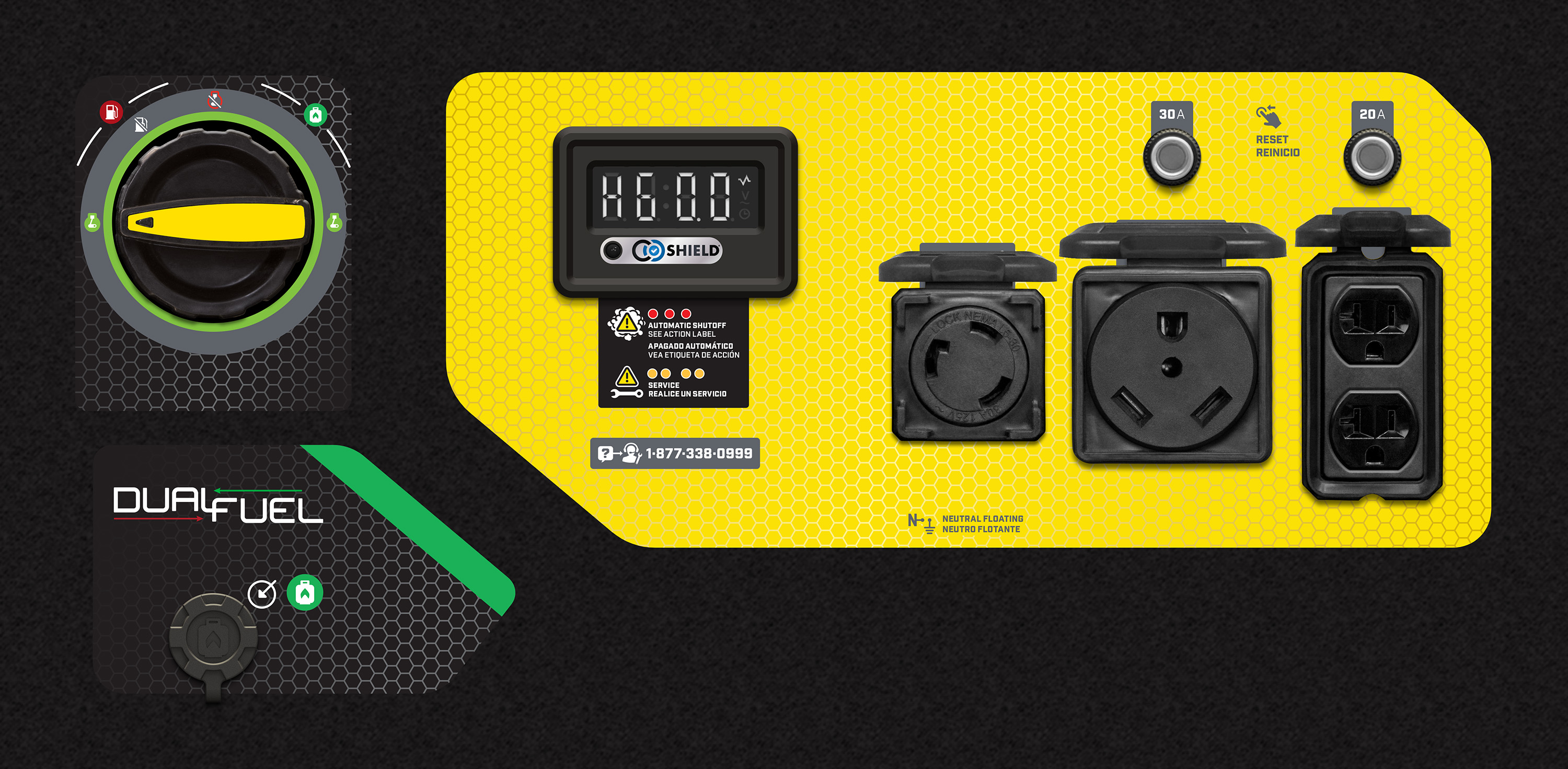Model: 201057
Cycles voltage, frequency (hertz), run time, and total run time, and power output.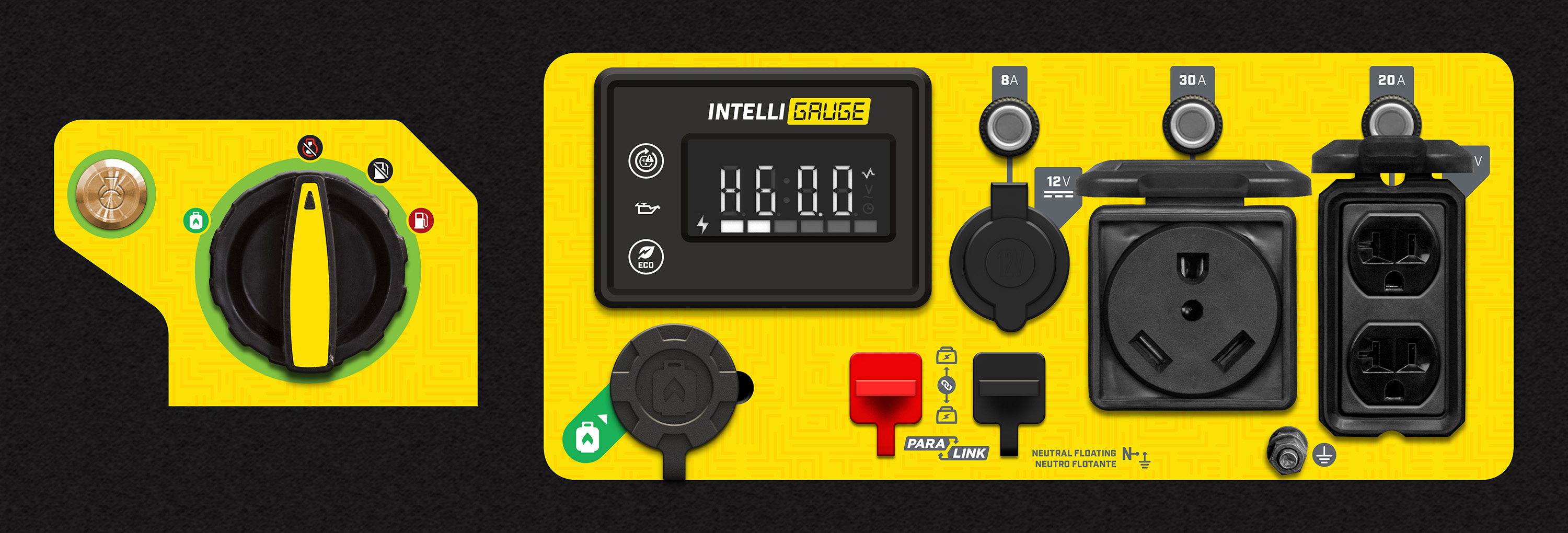Model: 200969, 200971
Cycles through frequency (hertz), voltage, and total run time.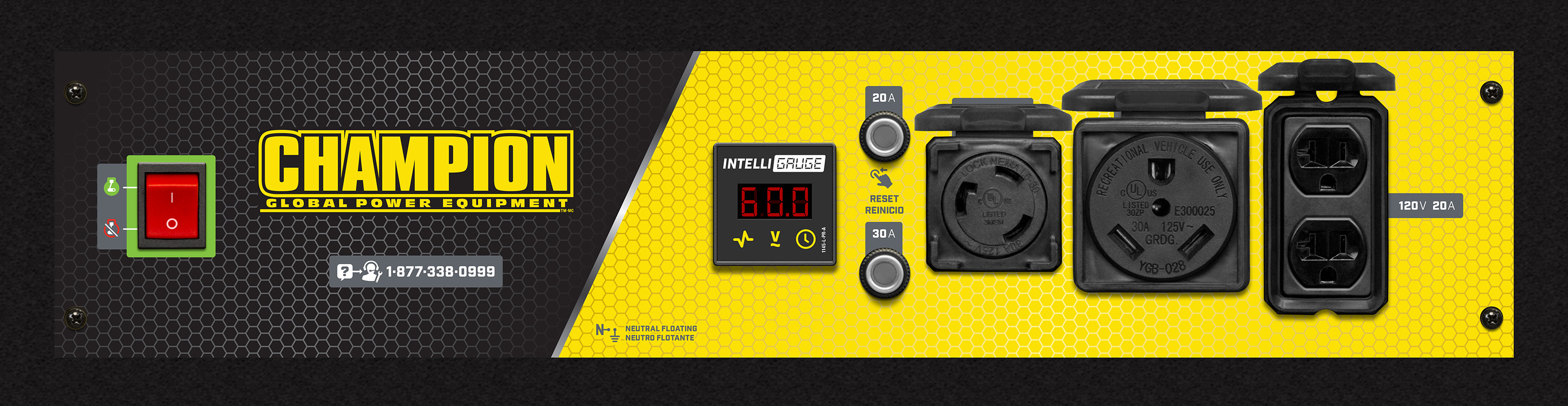3800-Watt Generators

Model: 76533
Cycles displaying running hours, voltage and hertz.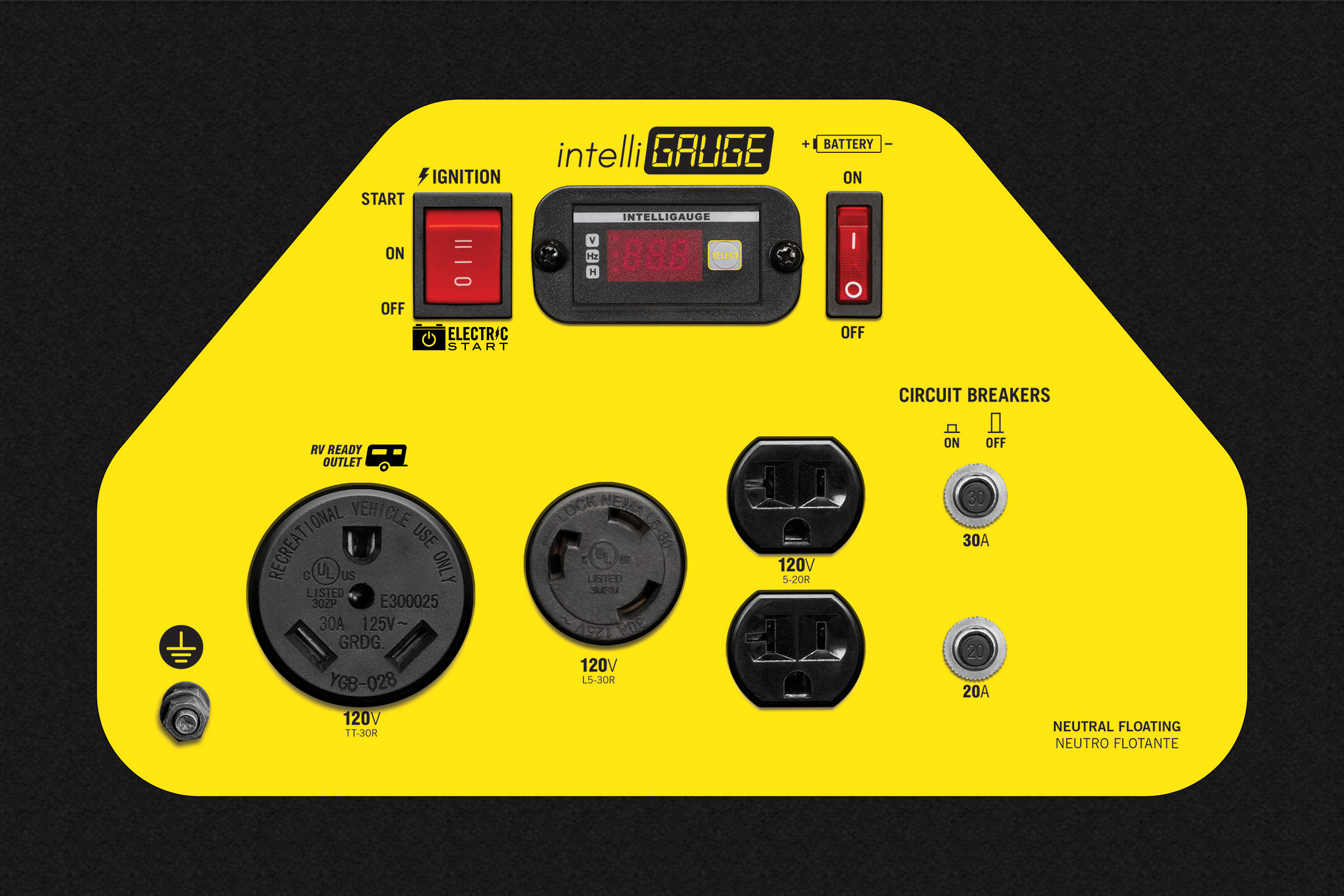Model: 201052
Cycles through voltage, frequency, run time, and total run time.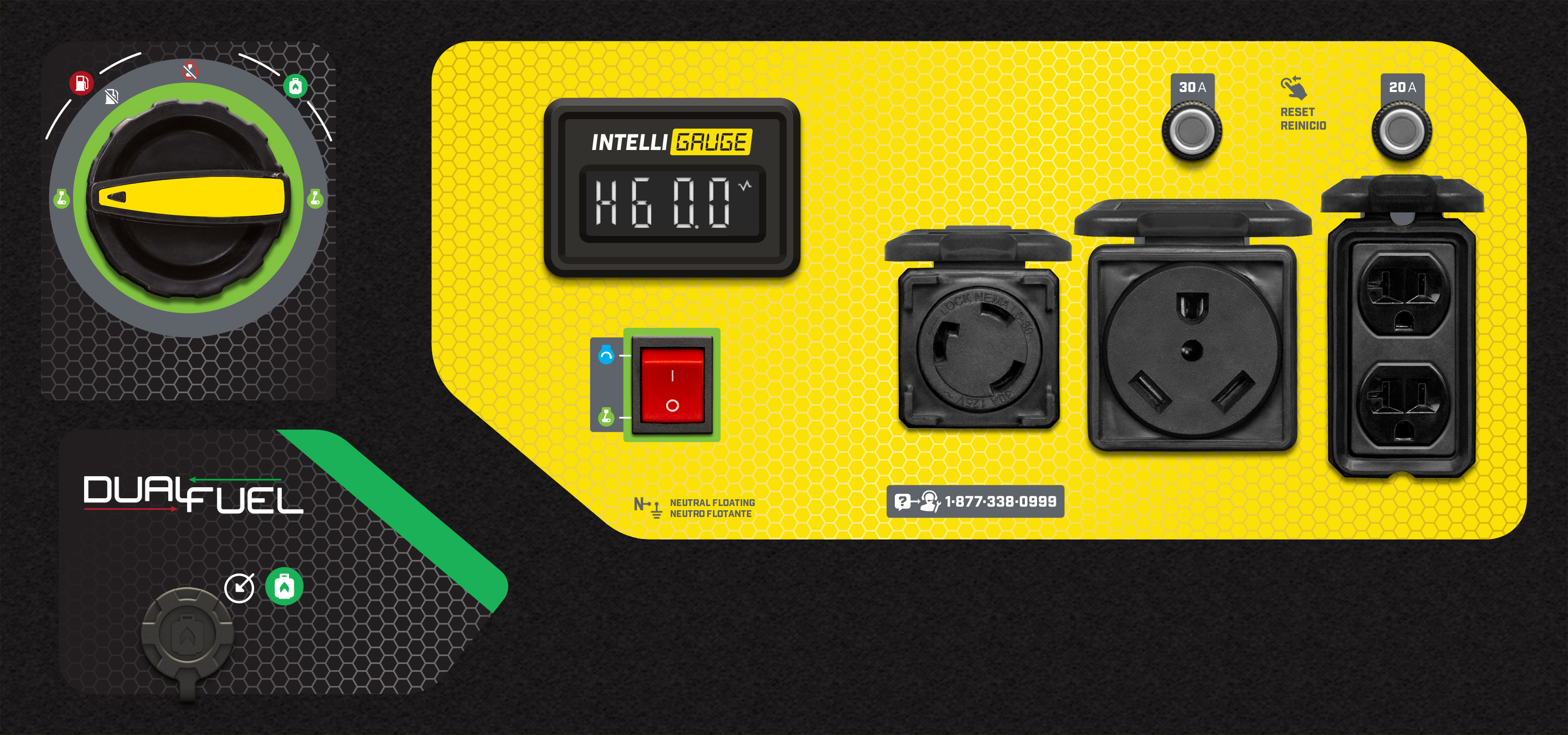4000-Watt Generators

Model: 200921
Cycles through frequency, voltage, and total run time.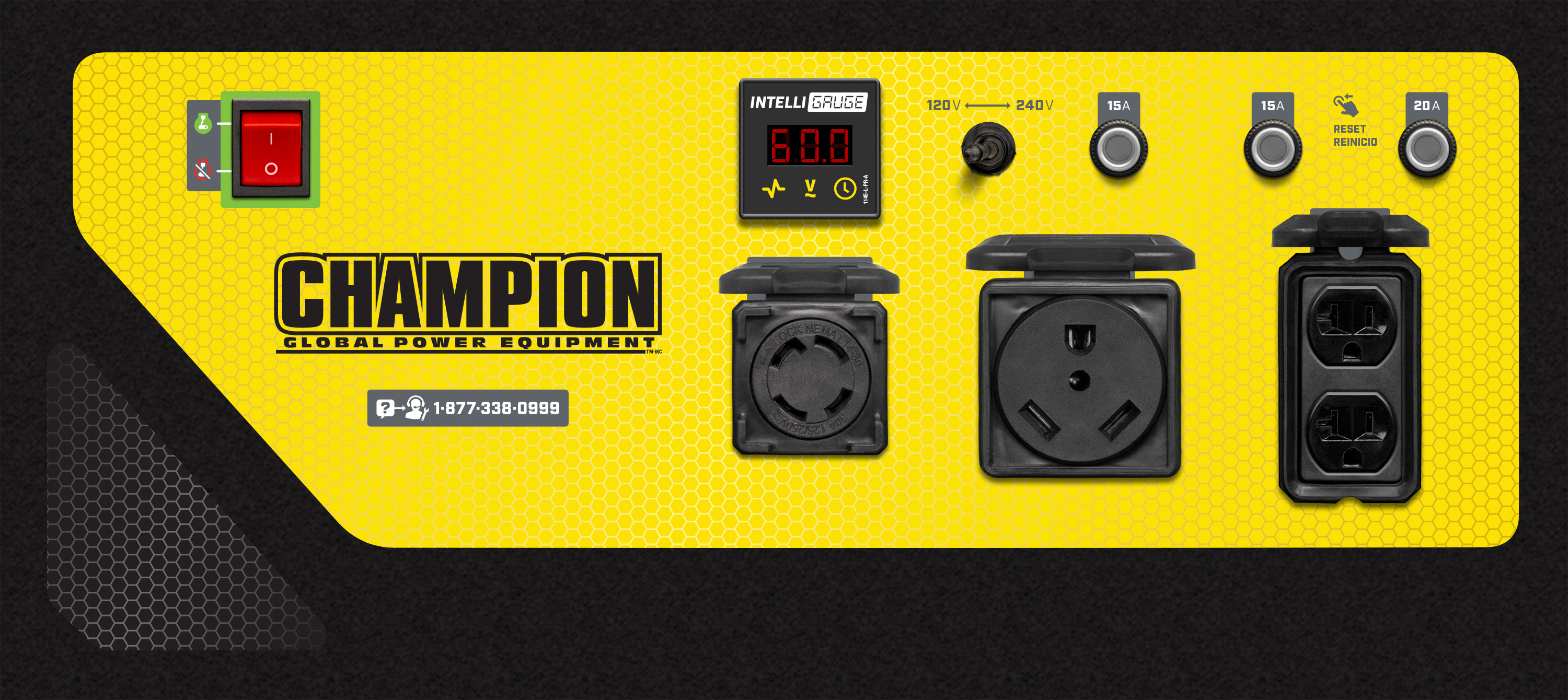4250-Watt Open Frame Inverters

Model: 200977, 200955, 200913
Cycles through frequency (hertz), voltage, and total run time.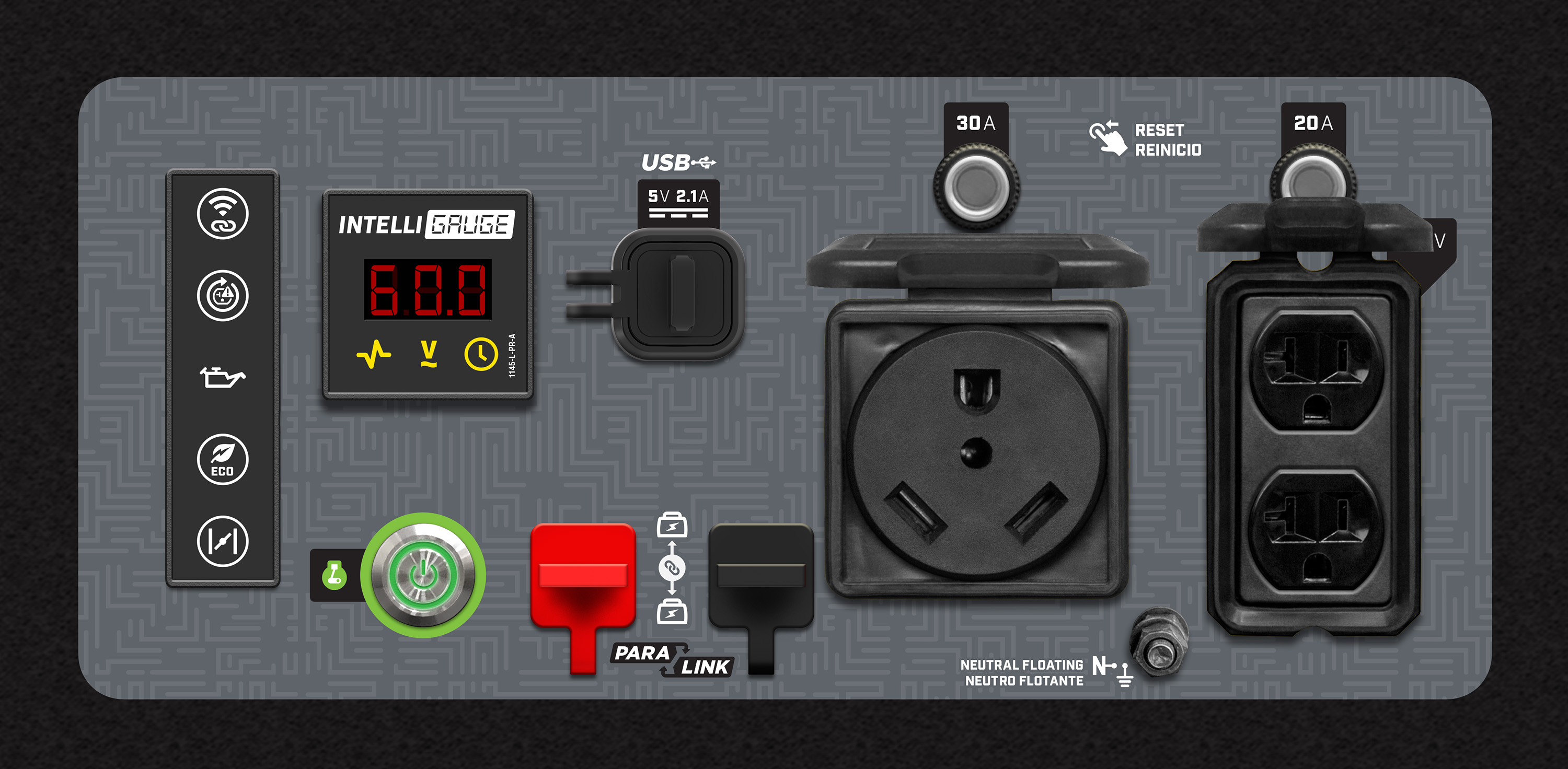Model: 201056, 201057
Cycles through voltage, frequency (hertz), run time, and total run time.Model: 200914, 200953, 200954
Cycles through voltage, frequency (hertz), run time, and total run time.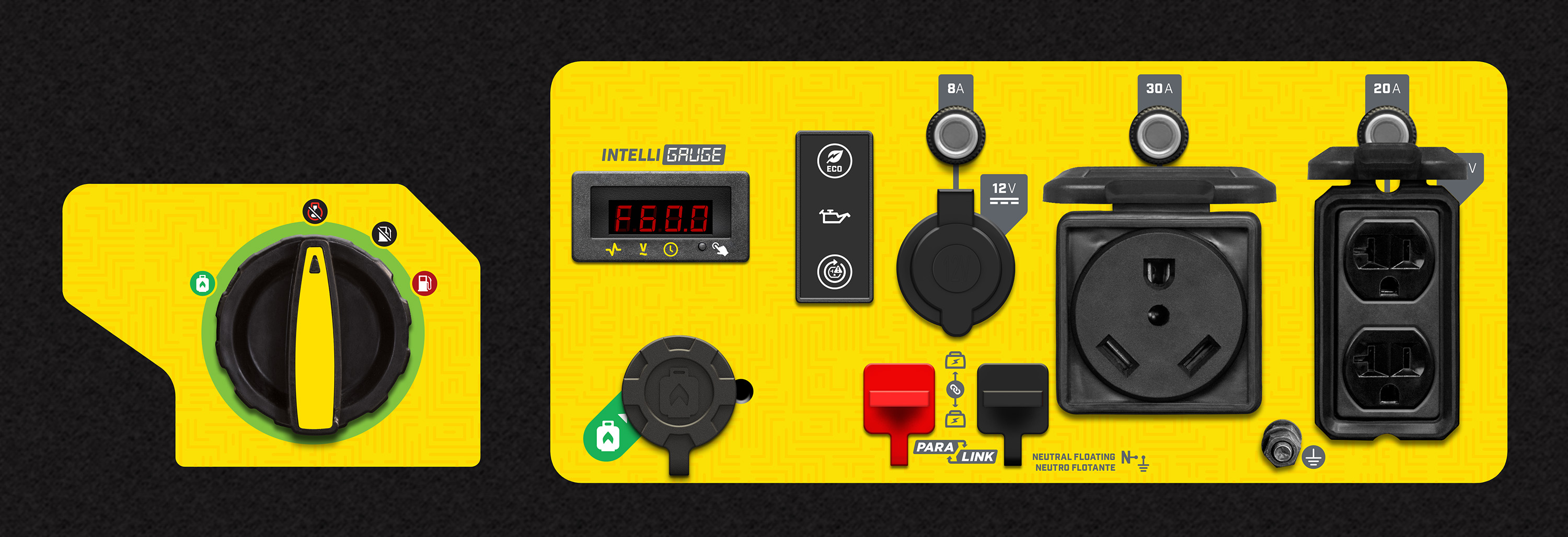4500-Watt Inverters

Model: 200986, 200987, 200988, 200989, 200990, 200991, 201053, 201064
Cycles through voltage, frequency, run time, and total run time.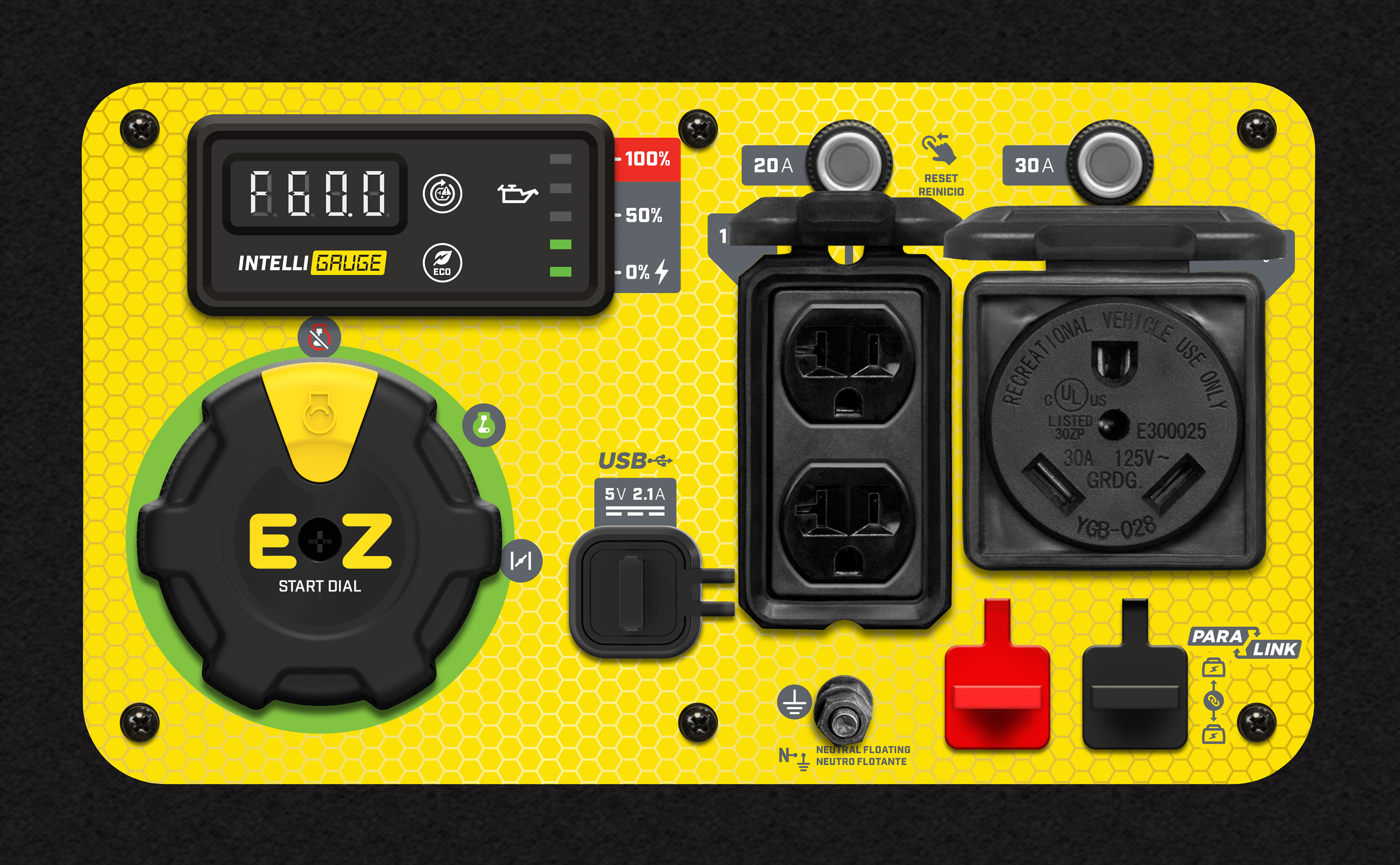Model: 200978, 201049
Cycles through voltage, frequency, run time, and total run time.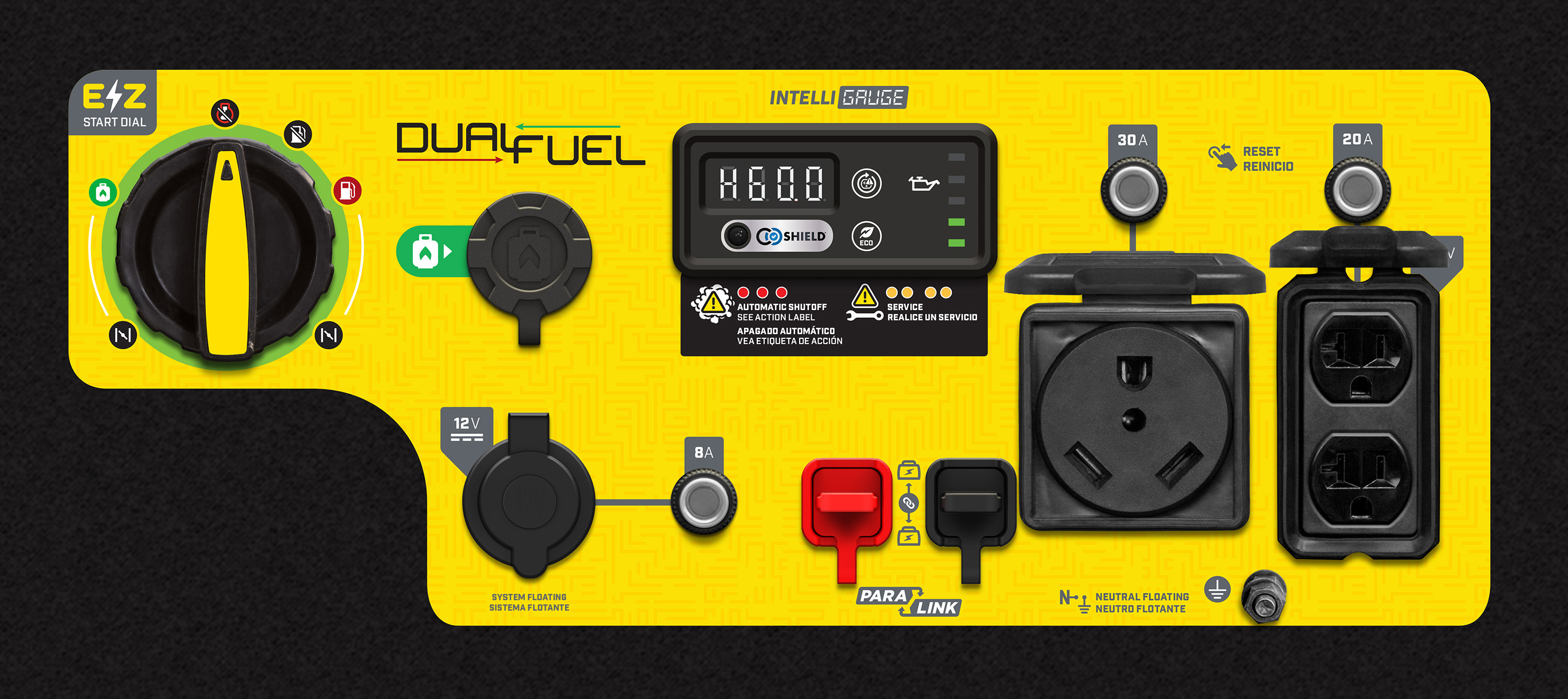Model: 201054
Cycles through voltage, frequency, run time, and total run time.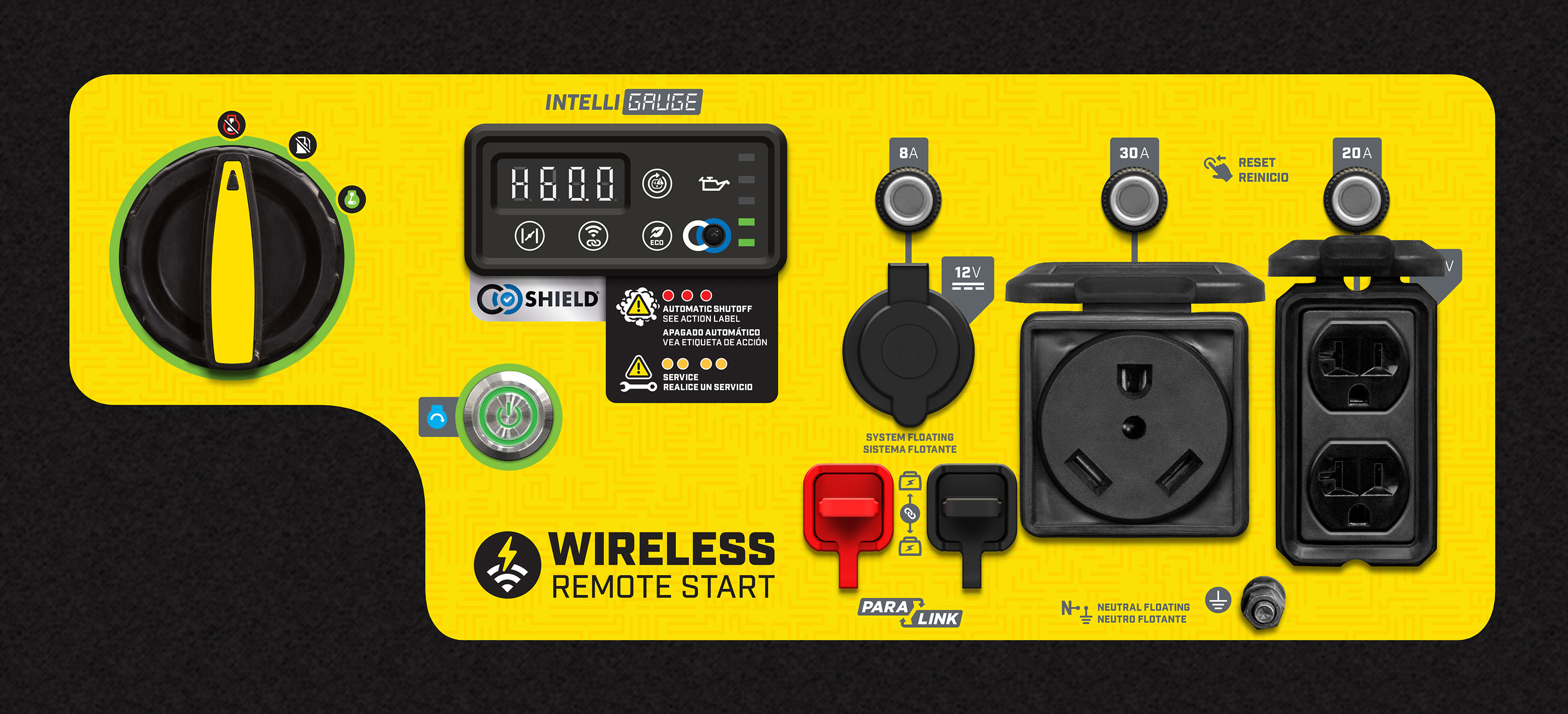Model: 100892
Cycles through frequency (hertz), voltage, and total run time.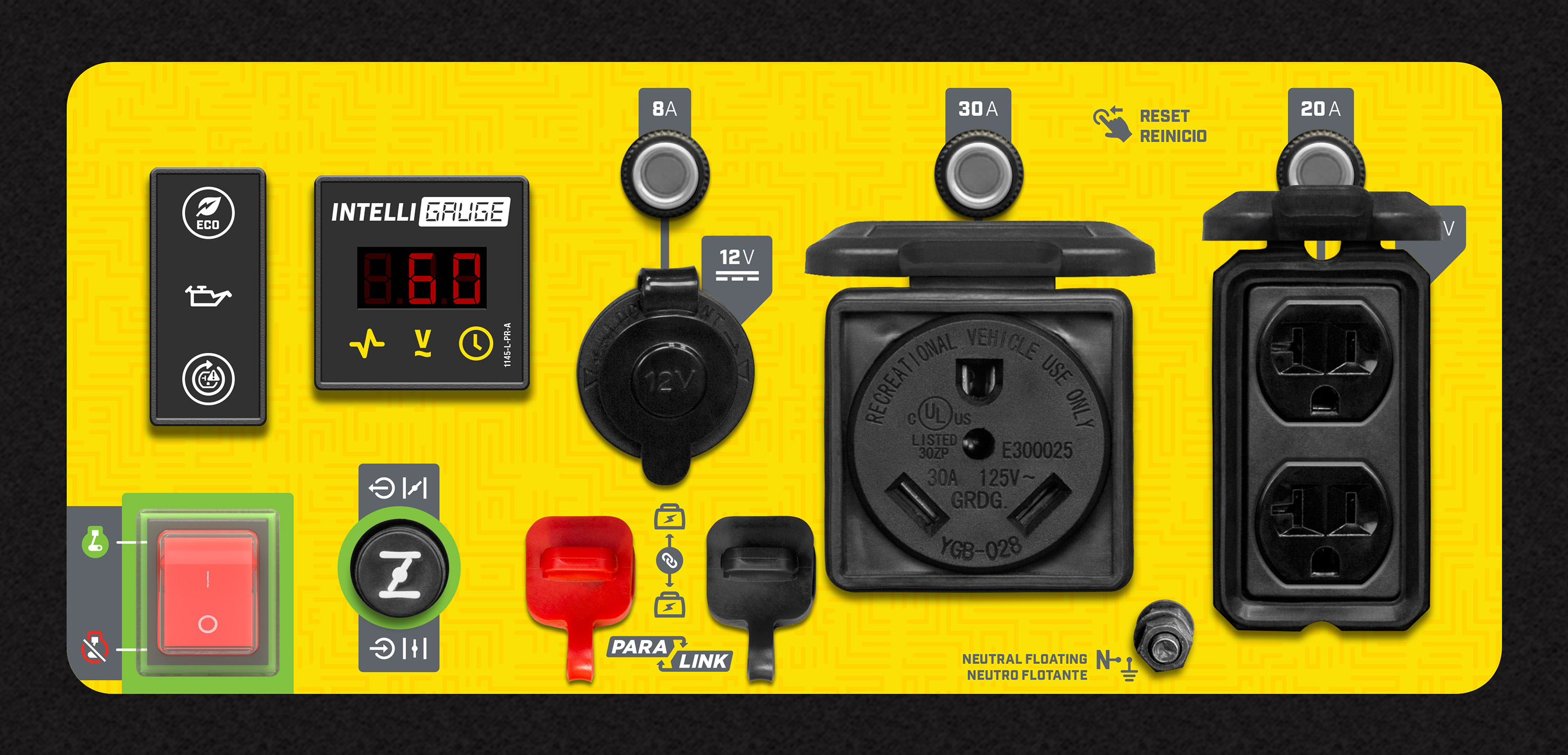4650-Watt Inverters

Model: 201080, 201120, 201154
Cycles through voltage, frequency, run time, and total run time.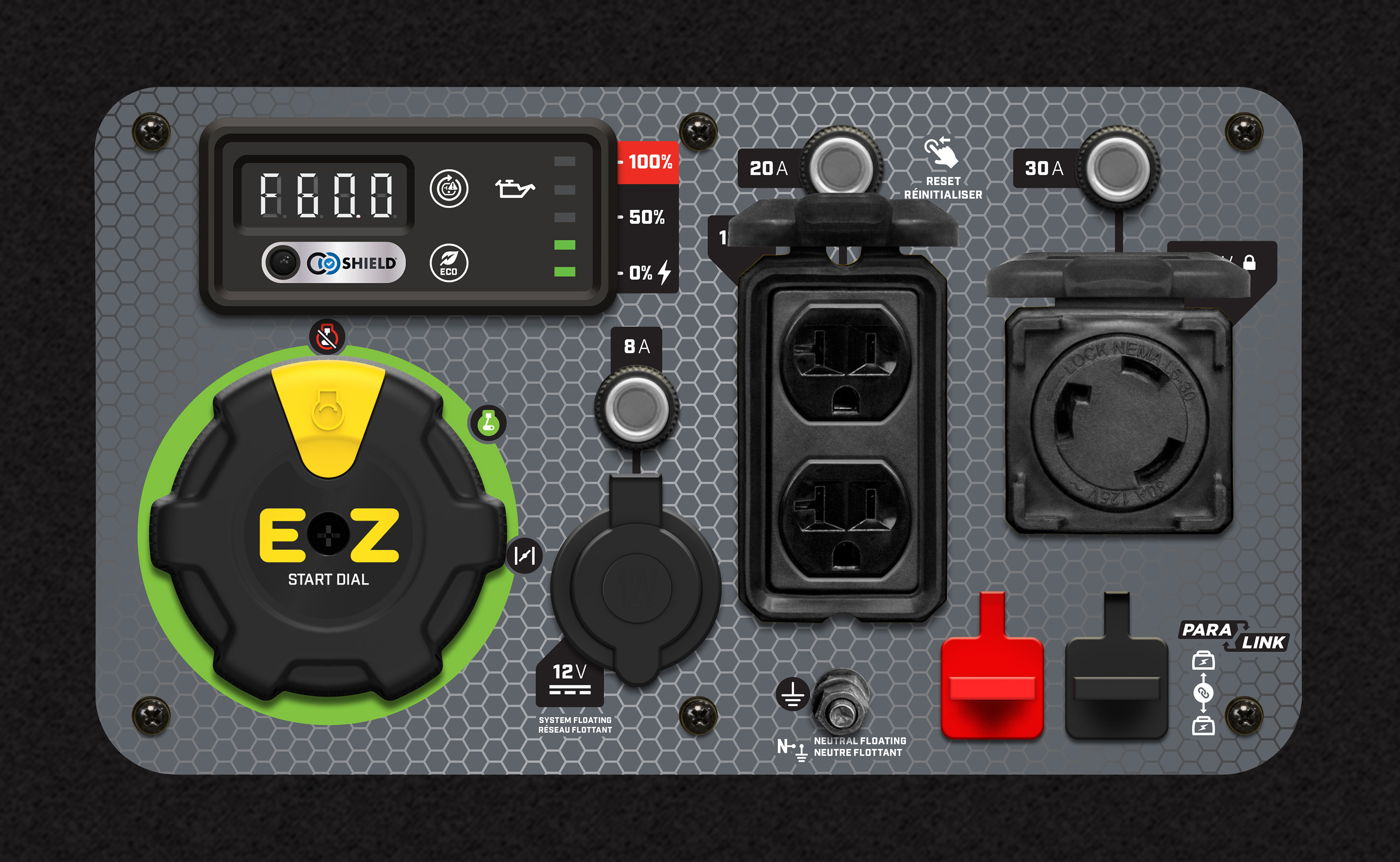Model: 200993
Cycles through voltage, frequency, run time, and total run time.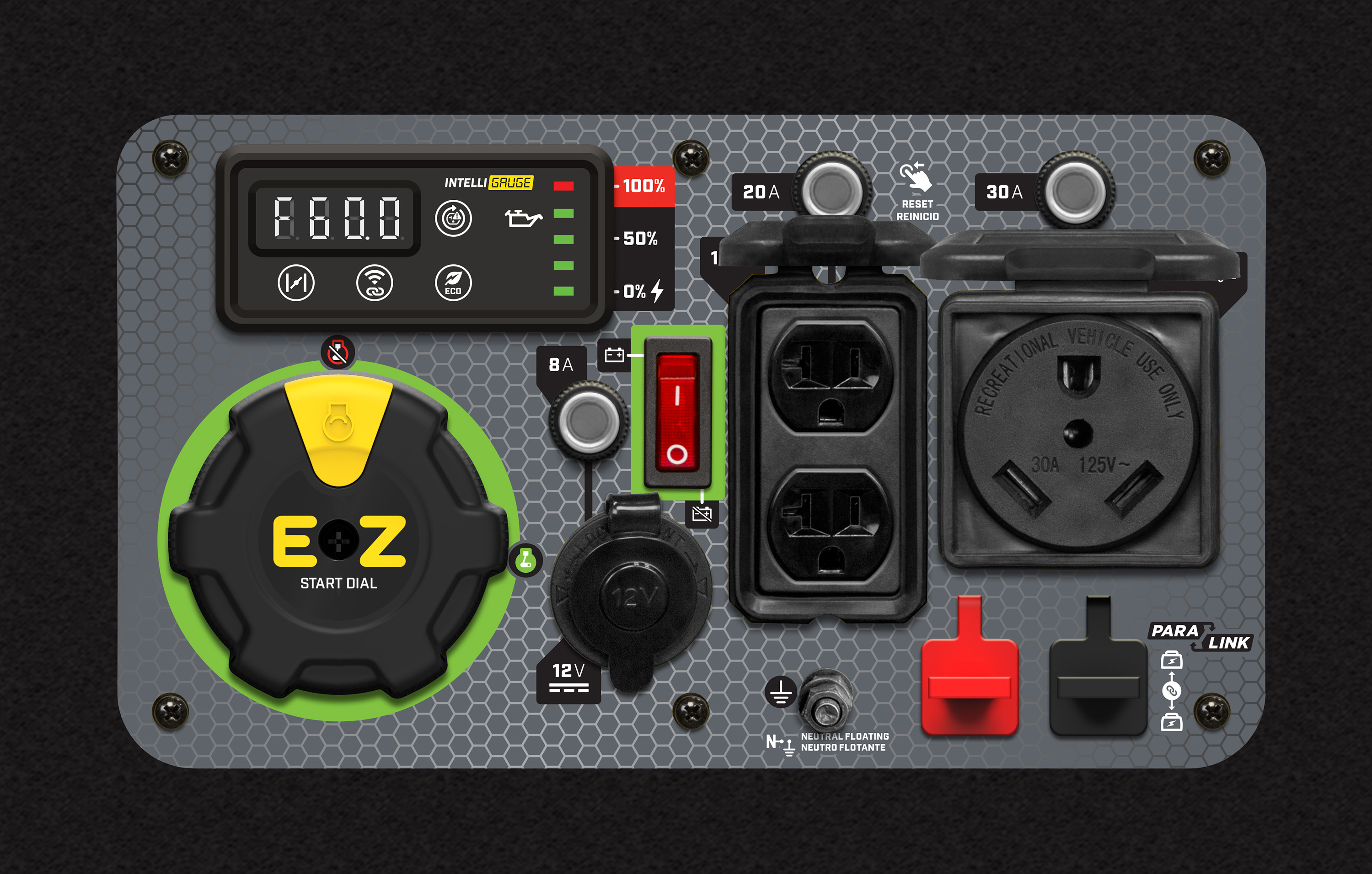Model: 201155
Cycles through voltage, frequency, run time, and total run time.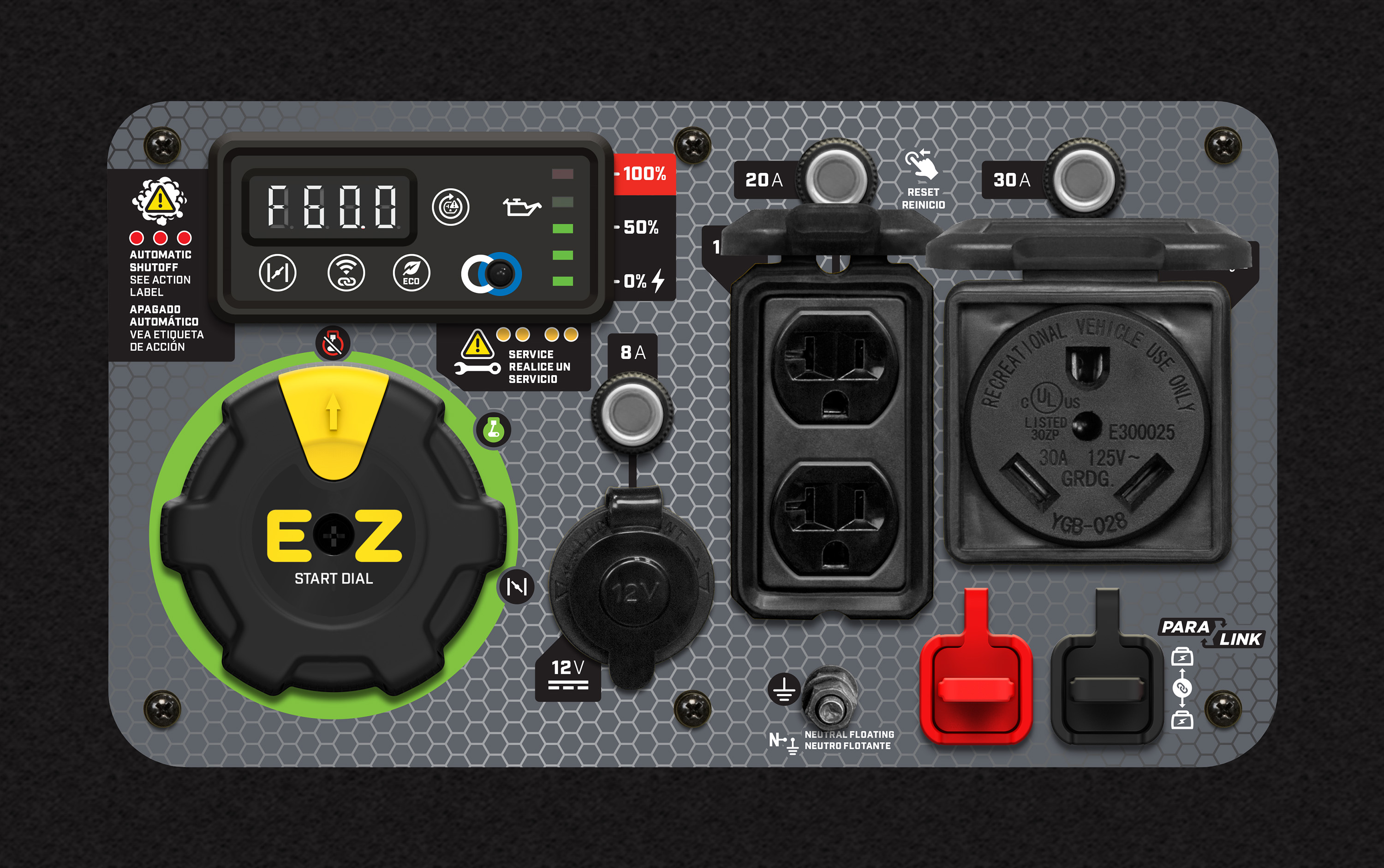Model: 200992, 200994
Cycles through voltage, frequency, run time, and total run time.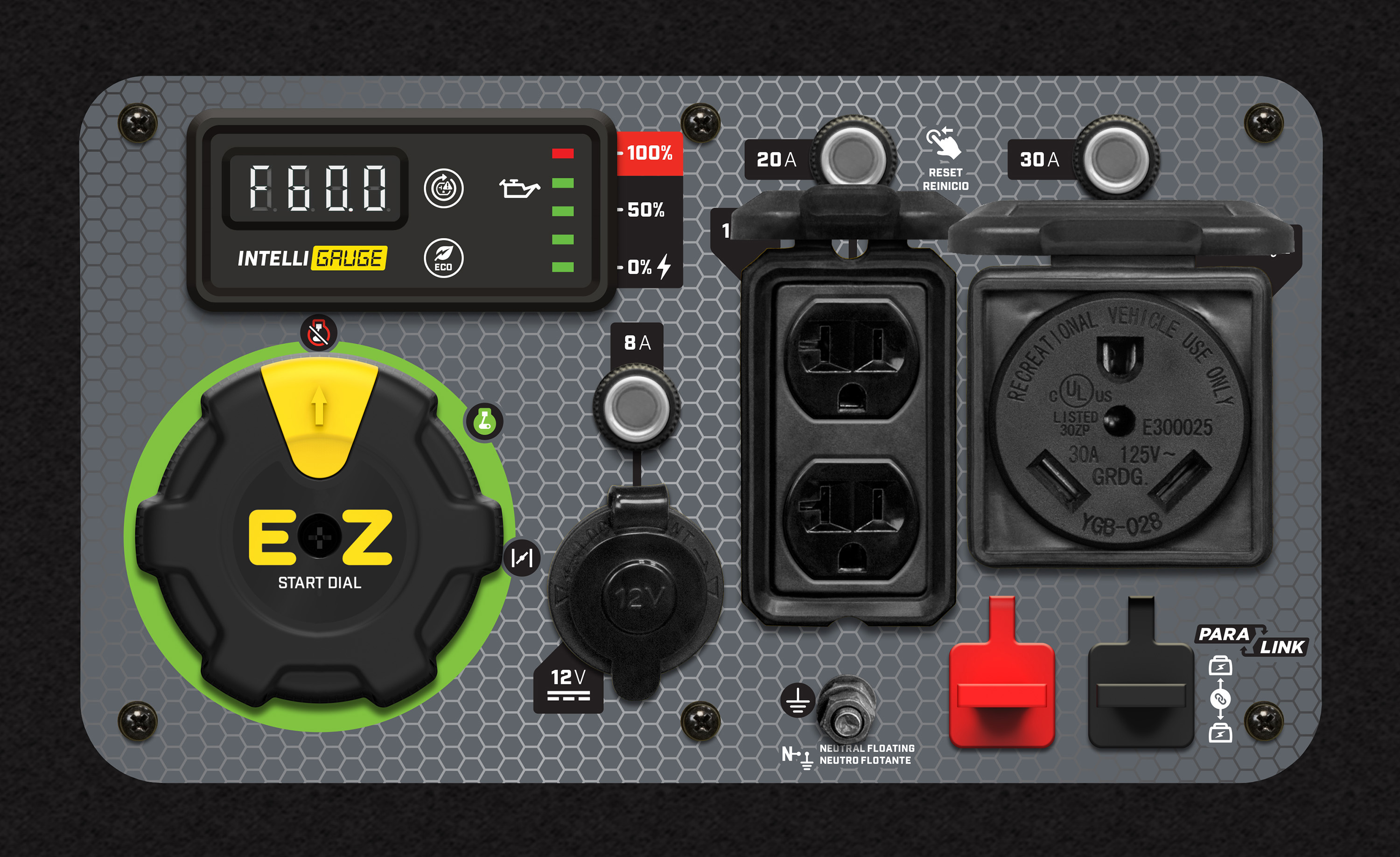5000-Watt Generators

Model: 100812
Cycles through running hours, voltage, and hertz.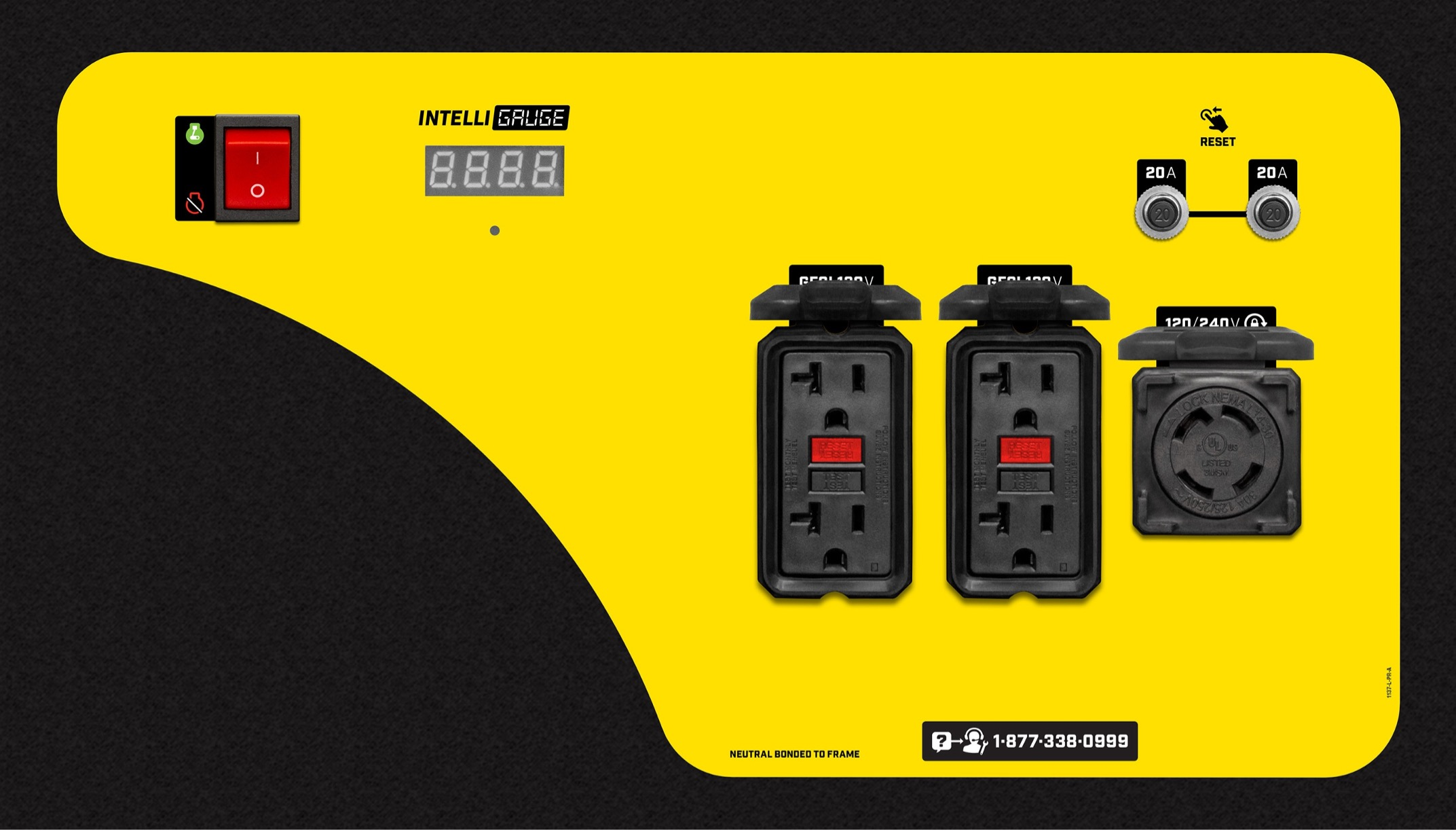Model: 201041
Cycles through voltage, frequency, run time, and total run time.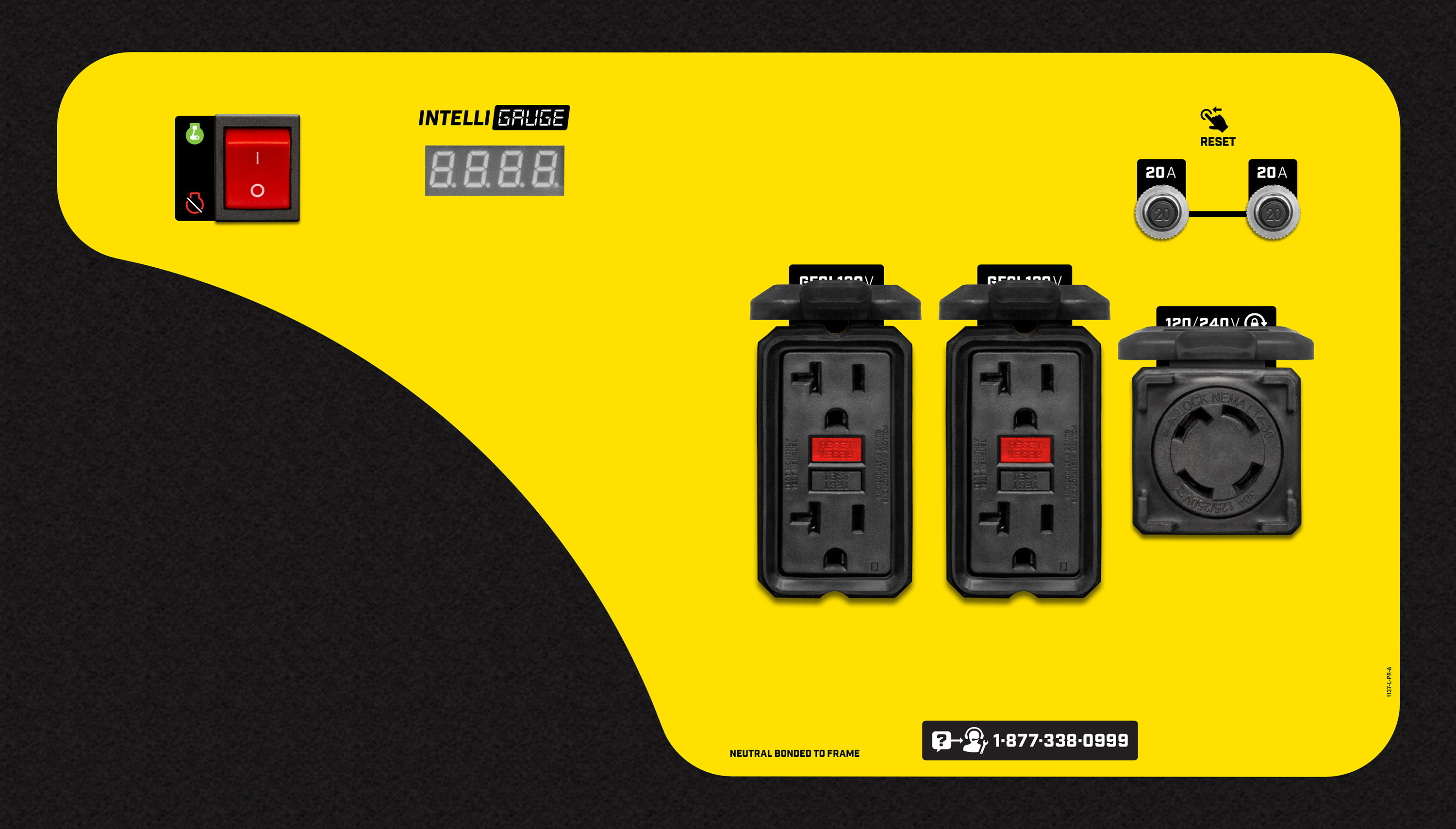5500-Watt Inverters

Model: 201001
Cycles through voltage, frequency (hertz), run time, total run time and power output.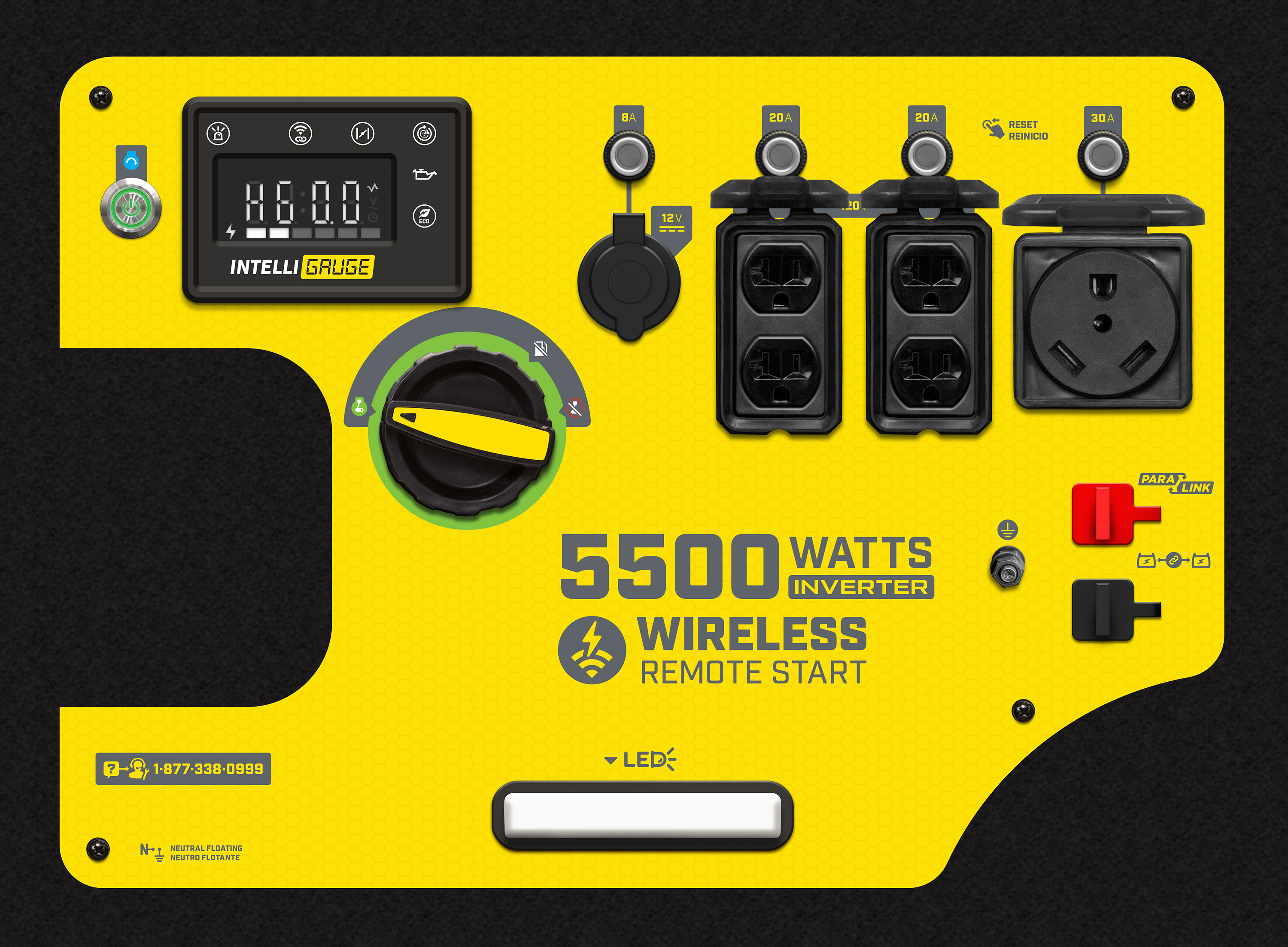5500-Watt Generators

Model: 100231
Cycles through voltage, hertz, and total run time.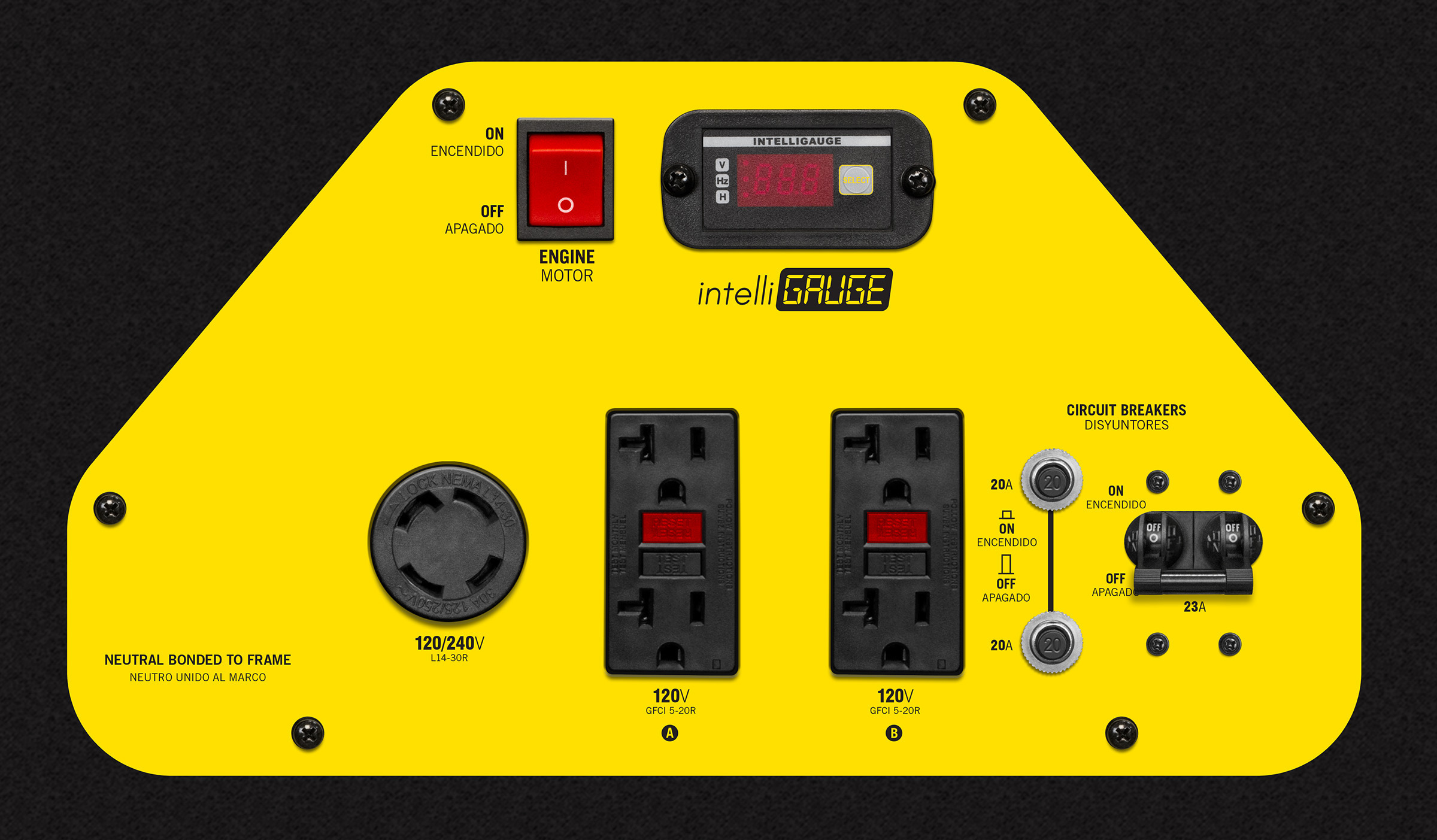Model: 201085
Cycles through voltage, frequency, session run time, total run time.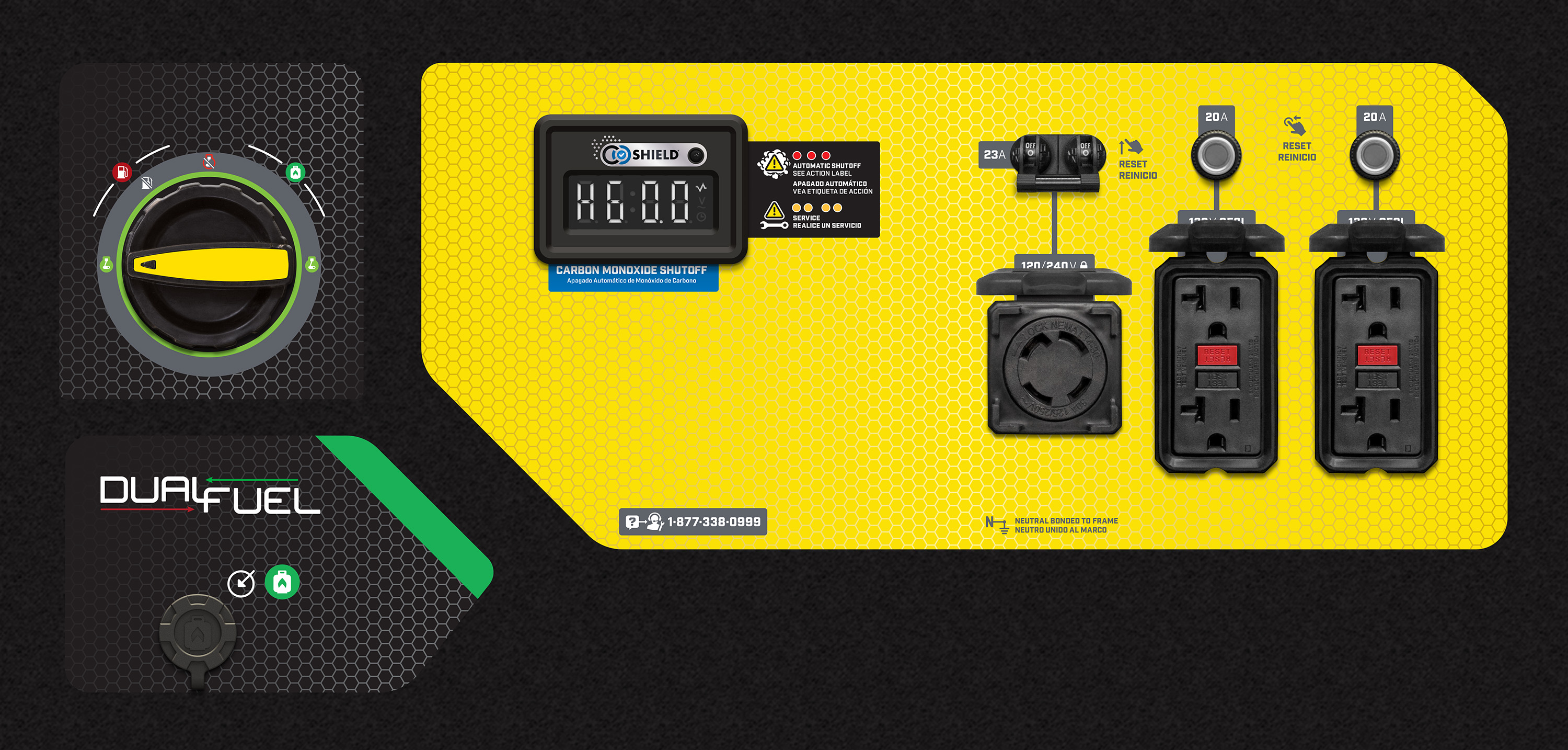Model: 201094
Cycles through voltage, frequency, run time, and total run time.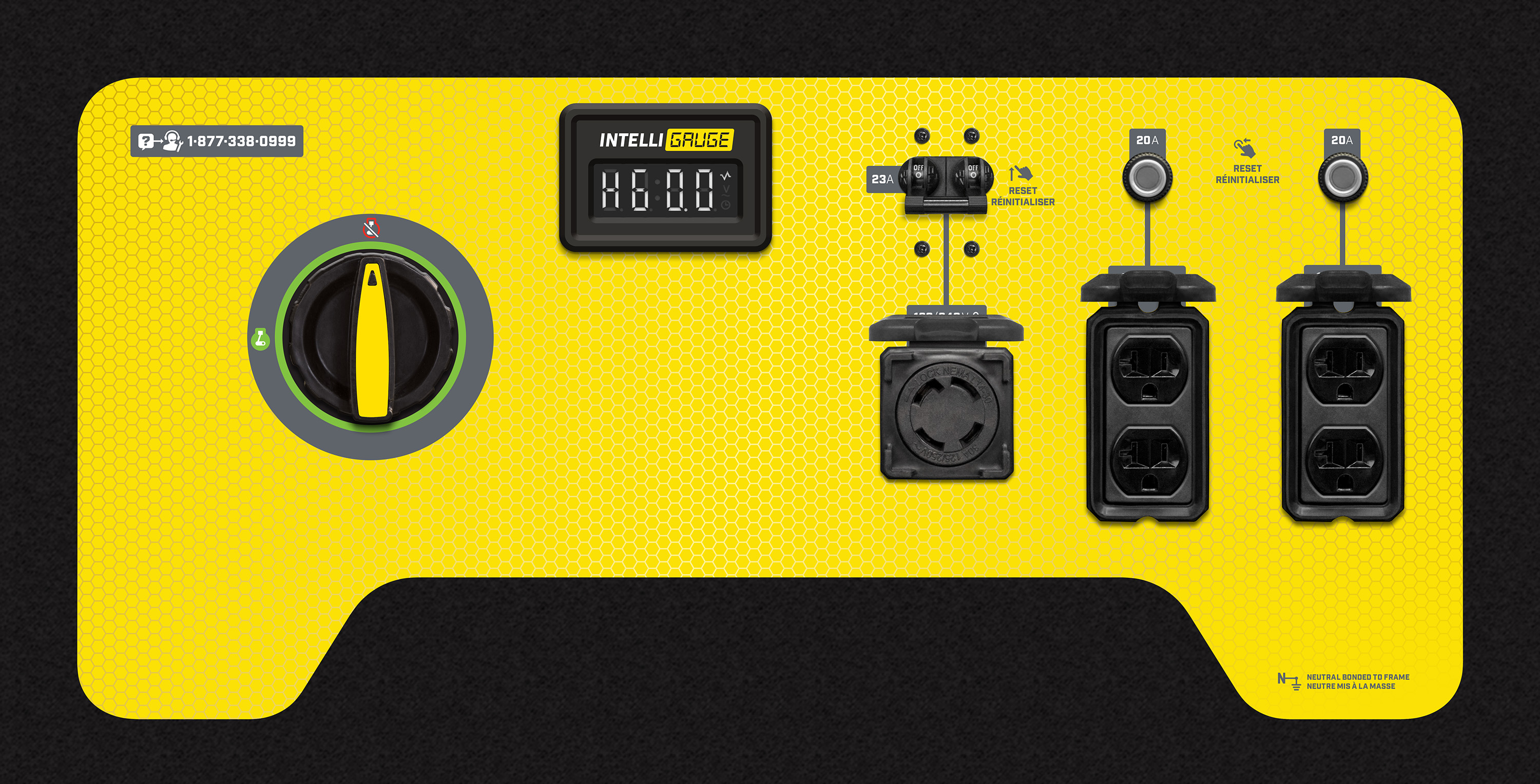6000-Watt Generators

Model: 201033
Cycles through run time, total run time, voltage, and hertz.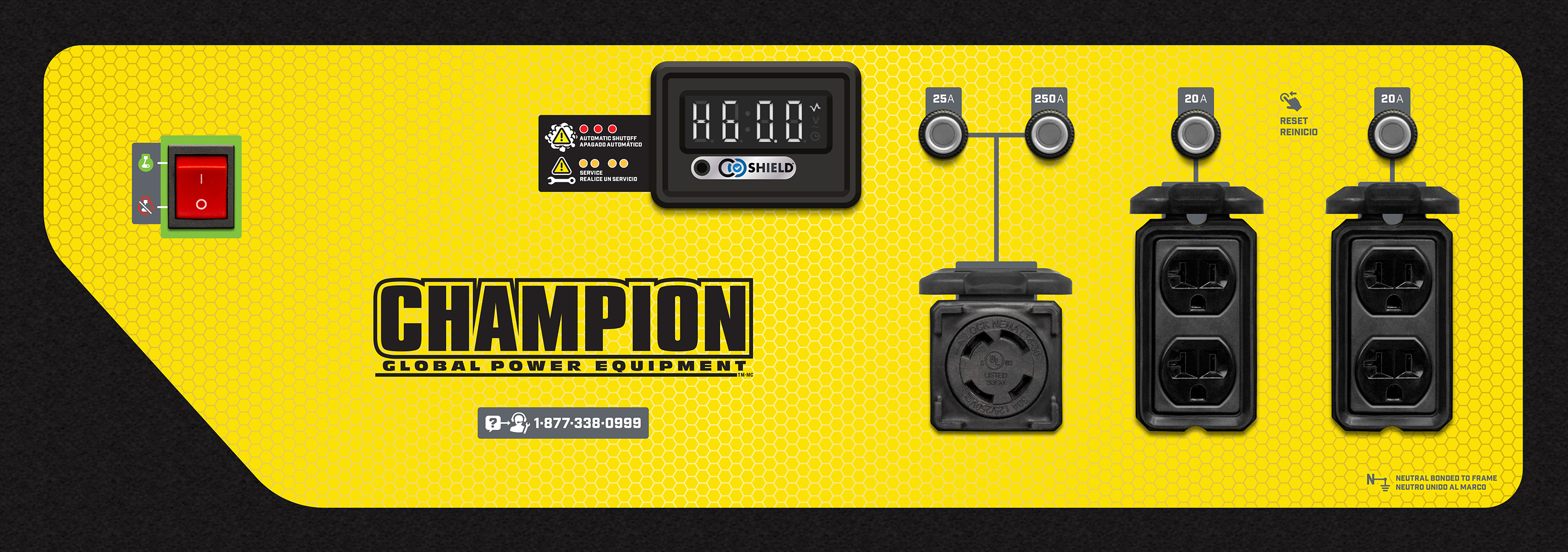6250-Watt Open Frame Inverters

Model: 100519
Cycles through voltage, frequency (hertz), run time, and total run time.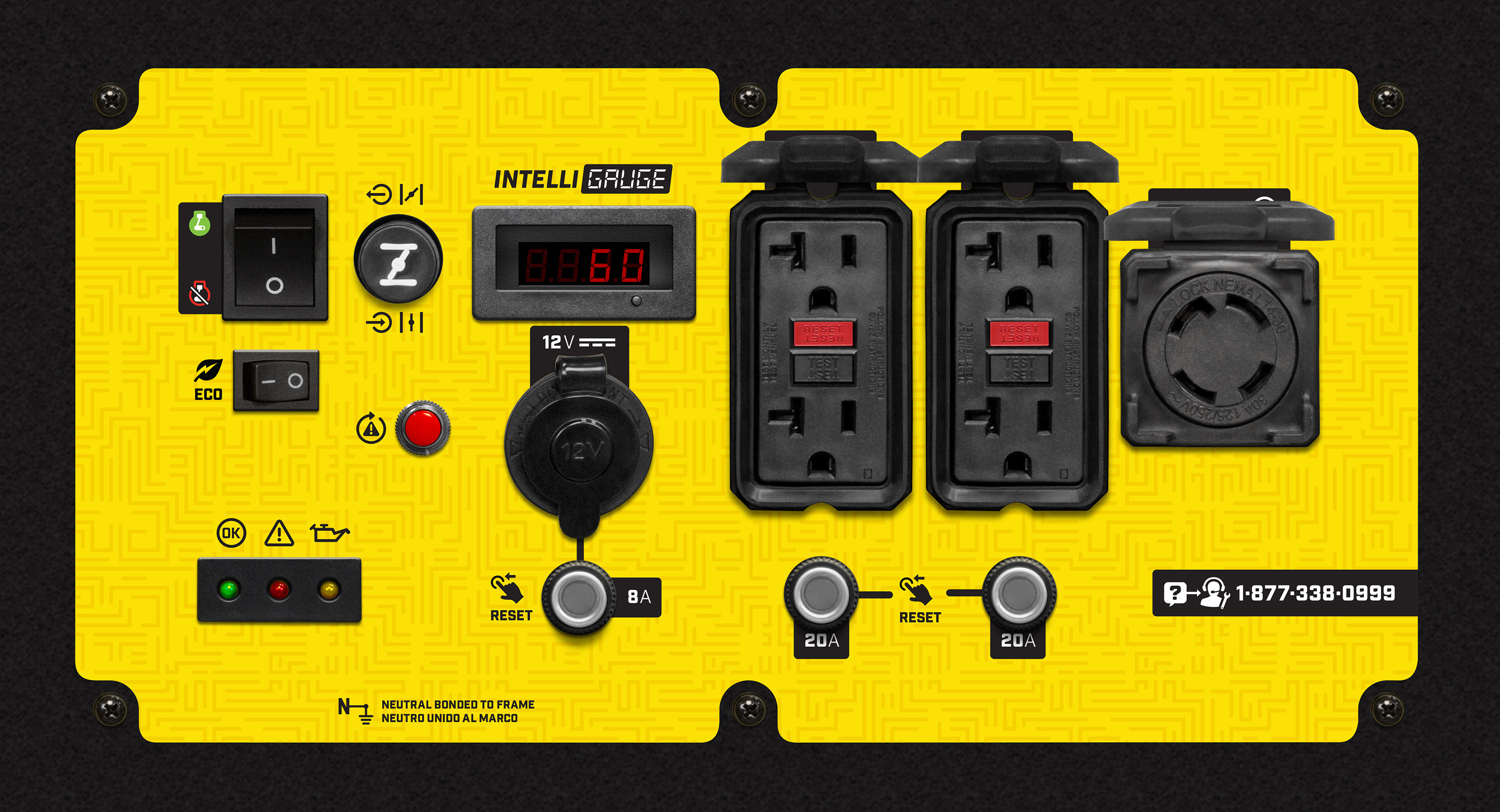6250-Watt Generators

Model: 100592
Cycles through voltage, hertz, and total run time.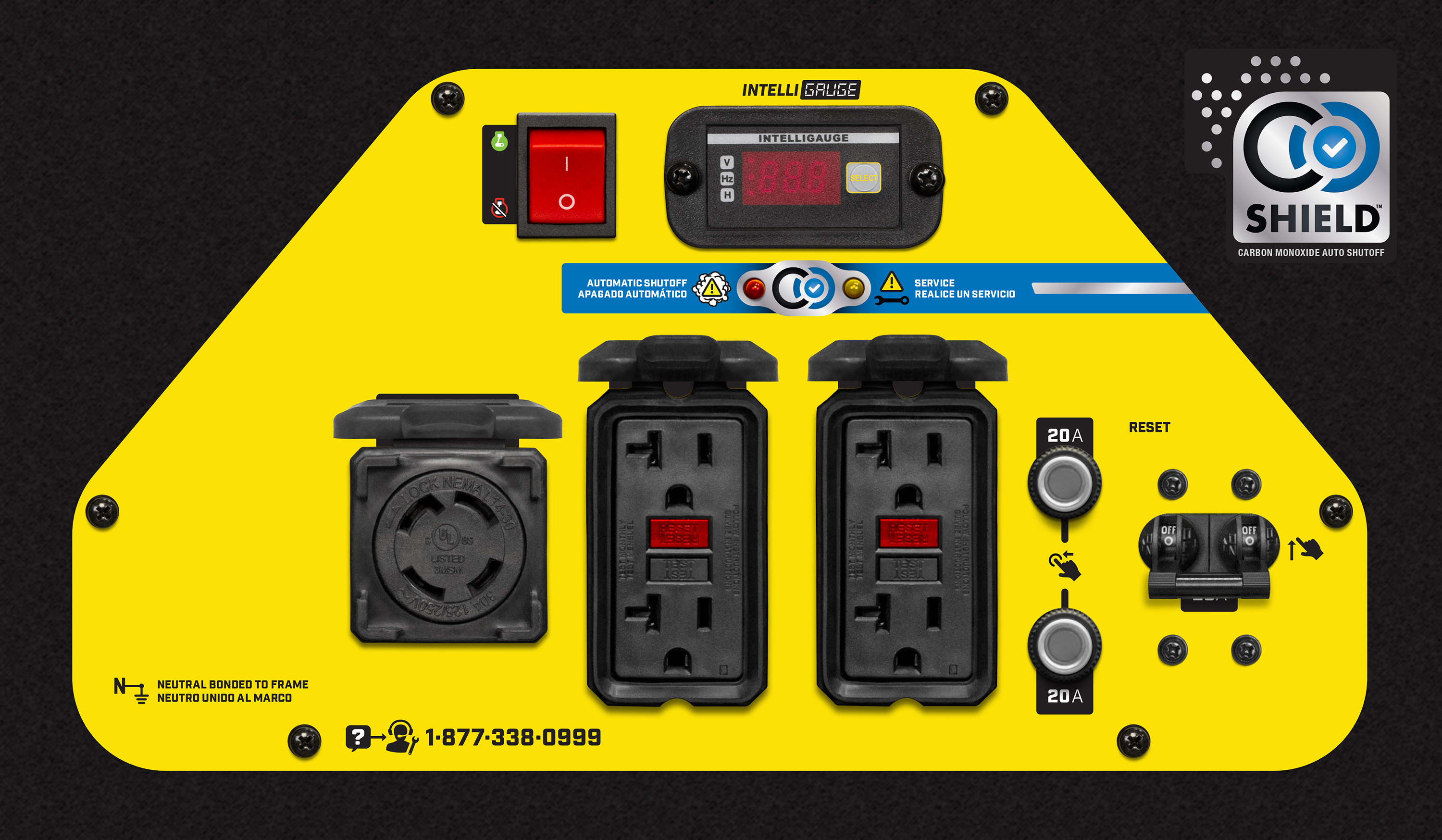Model: 100592V
Cycles through voltage, frequency, session run time, and total run time.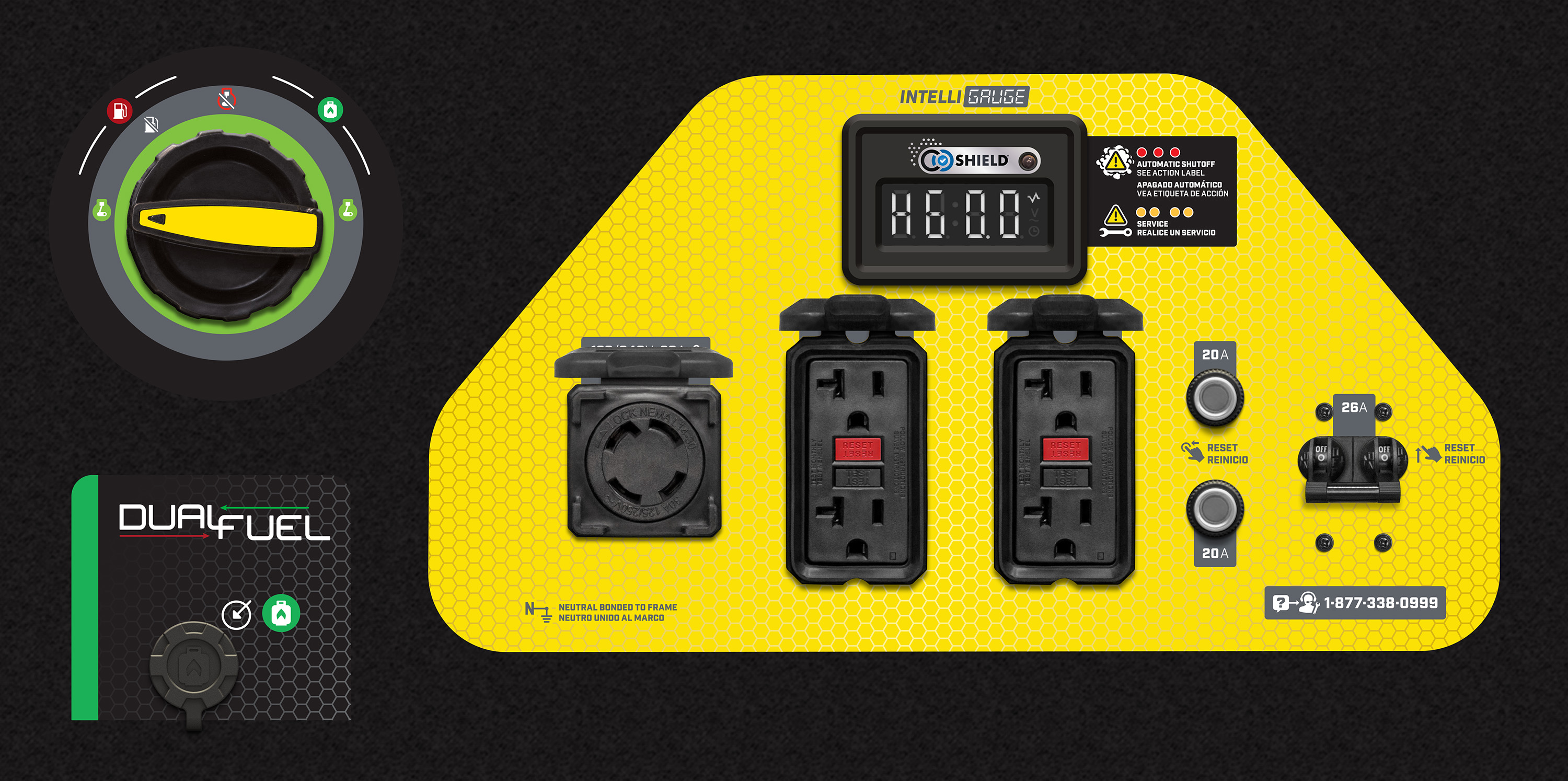6500-Watt Generators

Model: 100320
Cycles through running hours, voltage, and hertz.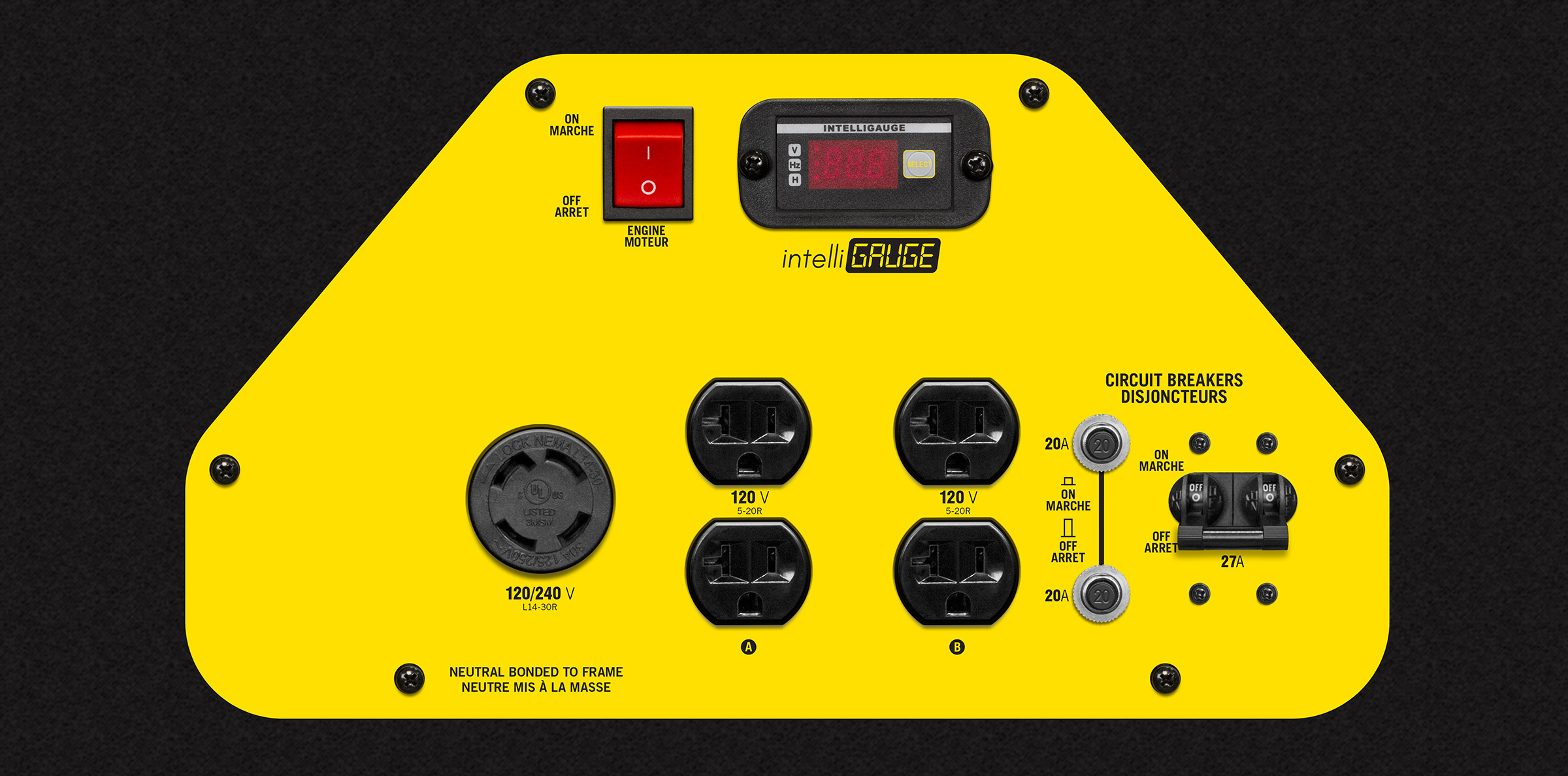Model: 100462
Cycles through run time, voltage, and hertz.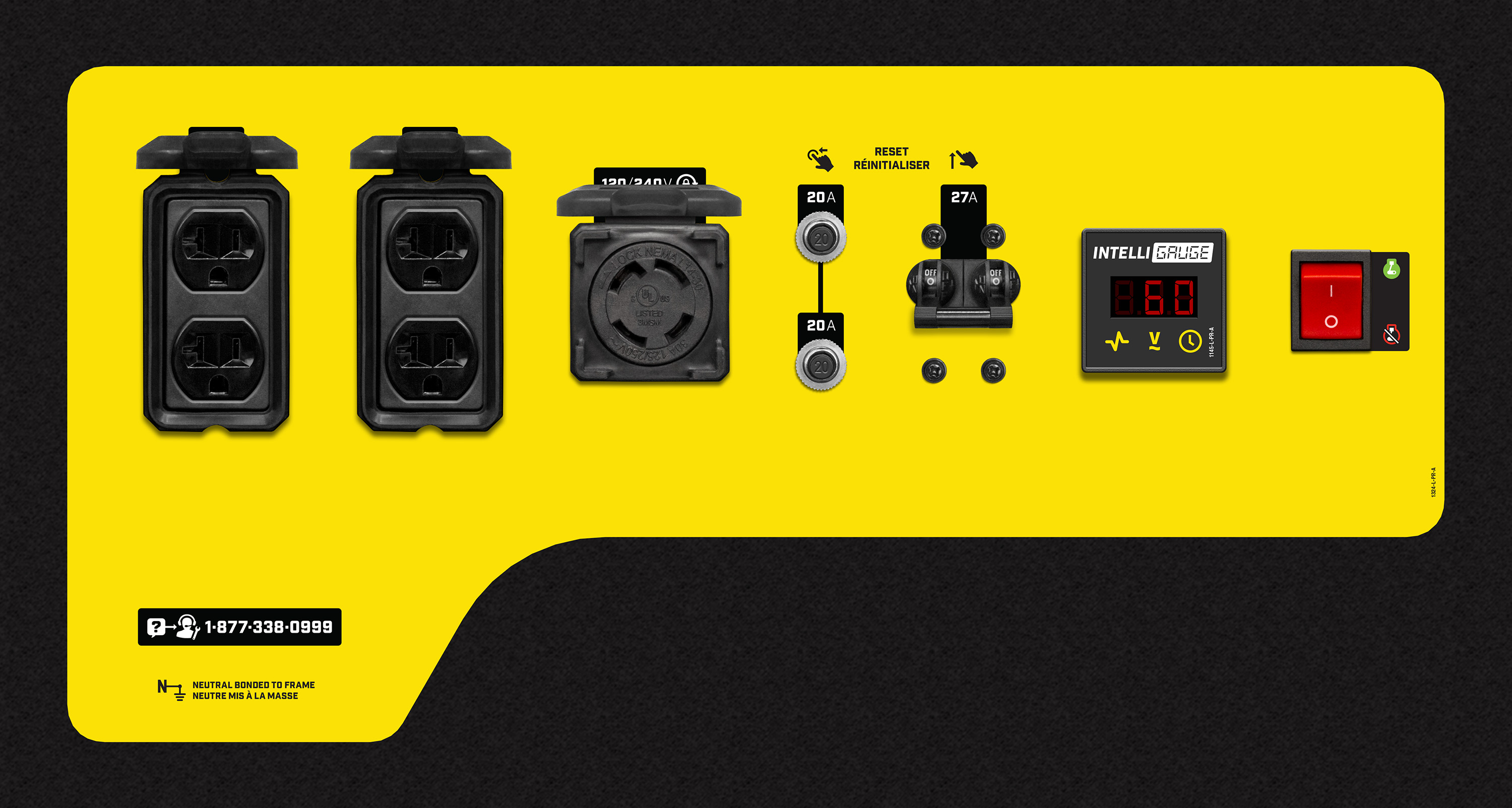7000-Watt Generators

Model: 201073
Cycles through voltage, frequency, run time, and total run time.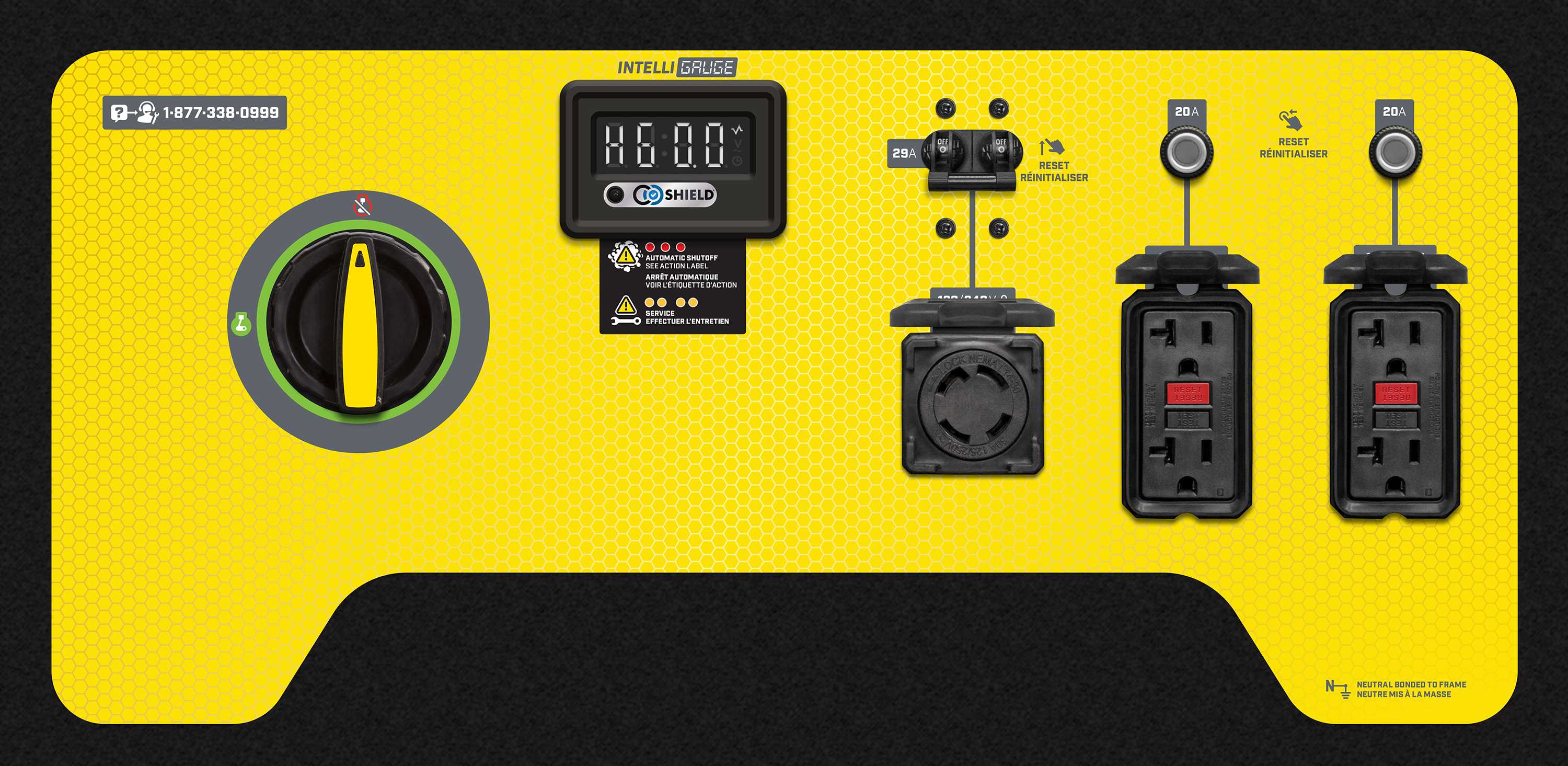7500-Watt Generators

Model: 100693, 100704, 100813, 100814, 201004
Cycles through voltage, frequency, run time, and total run time.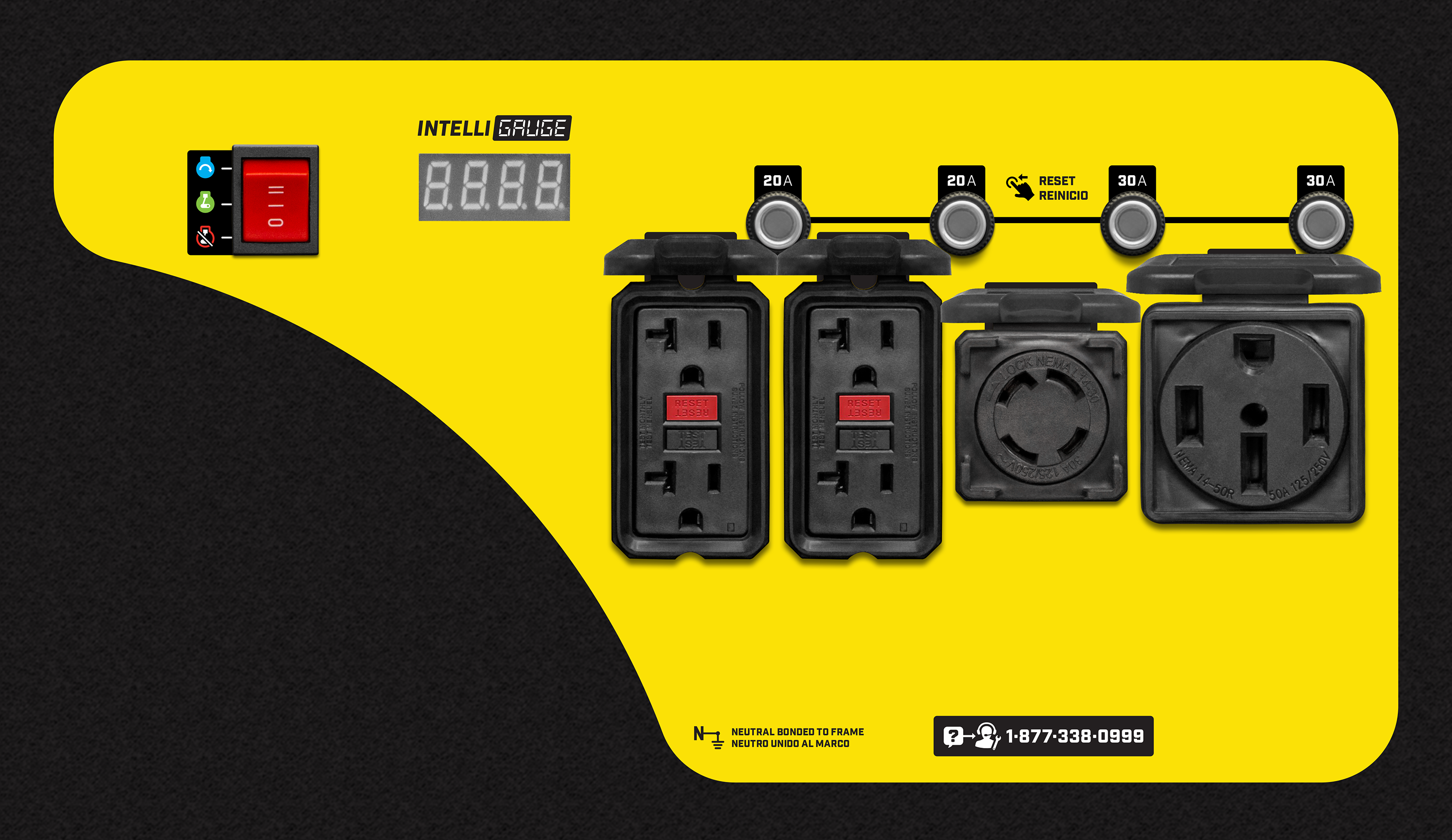Model: 100891, 201041
Cycles through voltage, frequency, running time, total run time, and power output.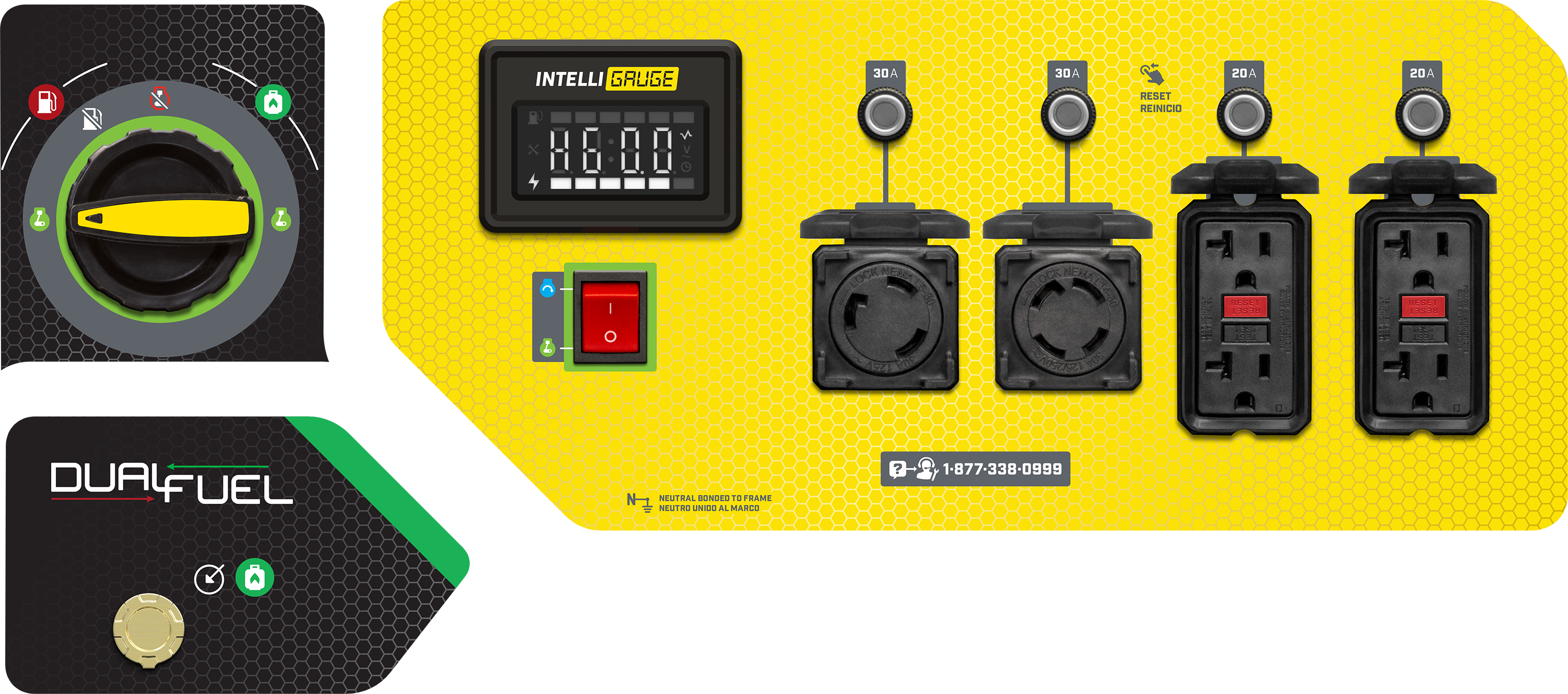Model: 100165, 100230, 100296
Cycles through total run time, voltage, and hertz.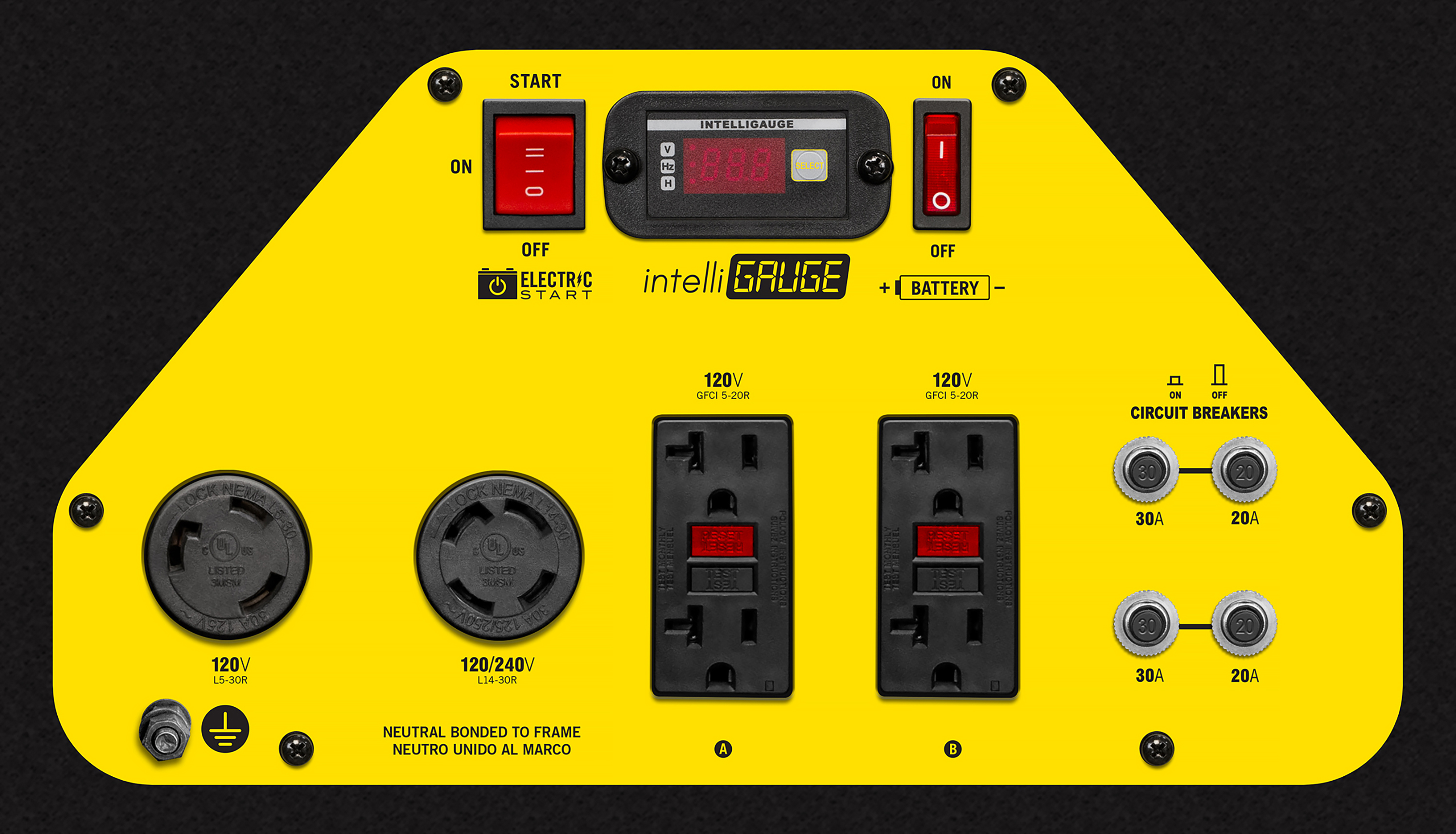Model: 100463
Cycles through run time, voltage, and hertz.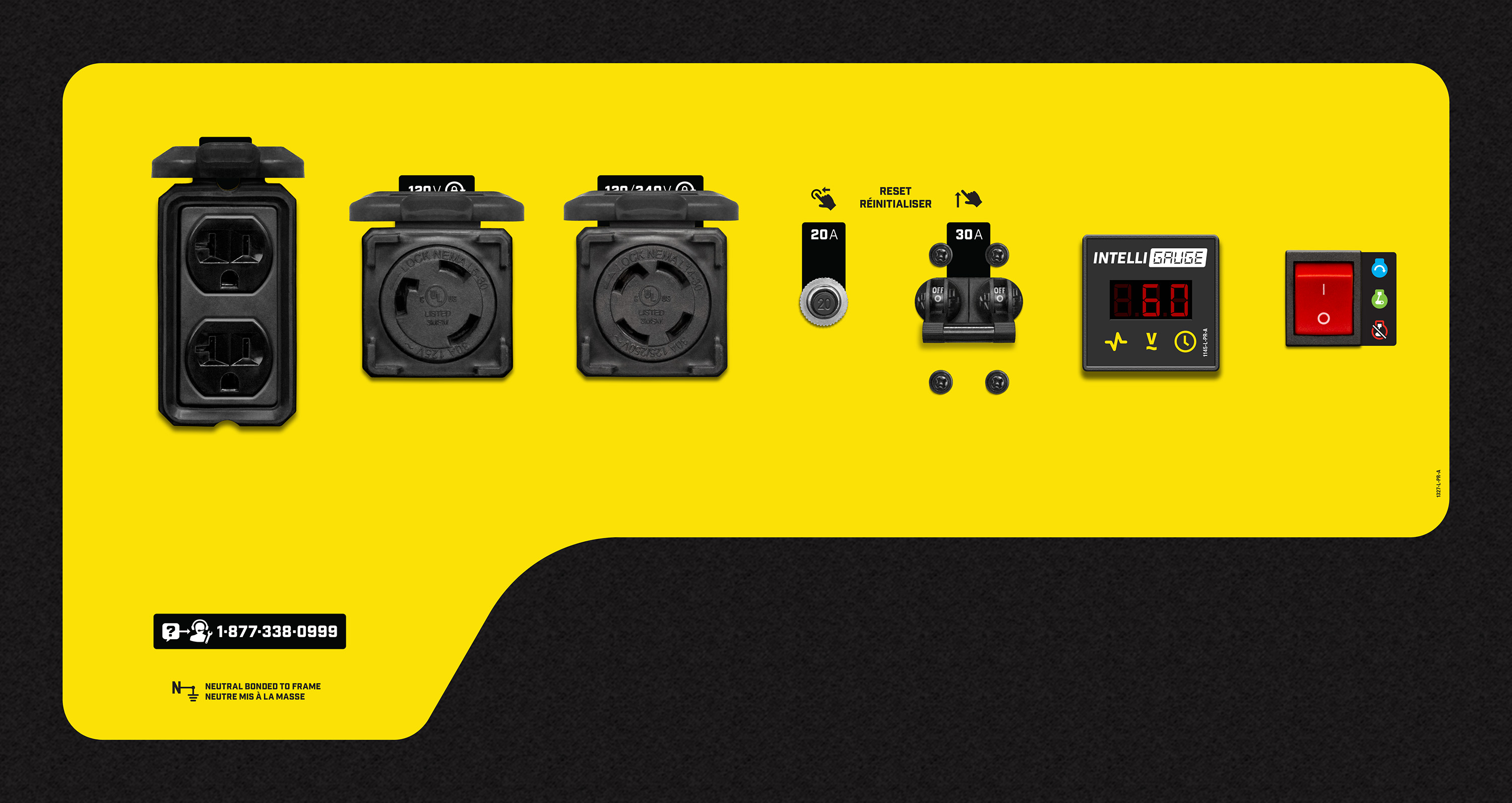Model: 201158
Cycles through voltage, frequency, run time, and total run time.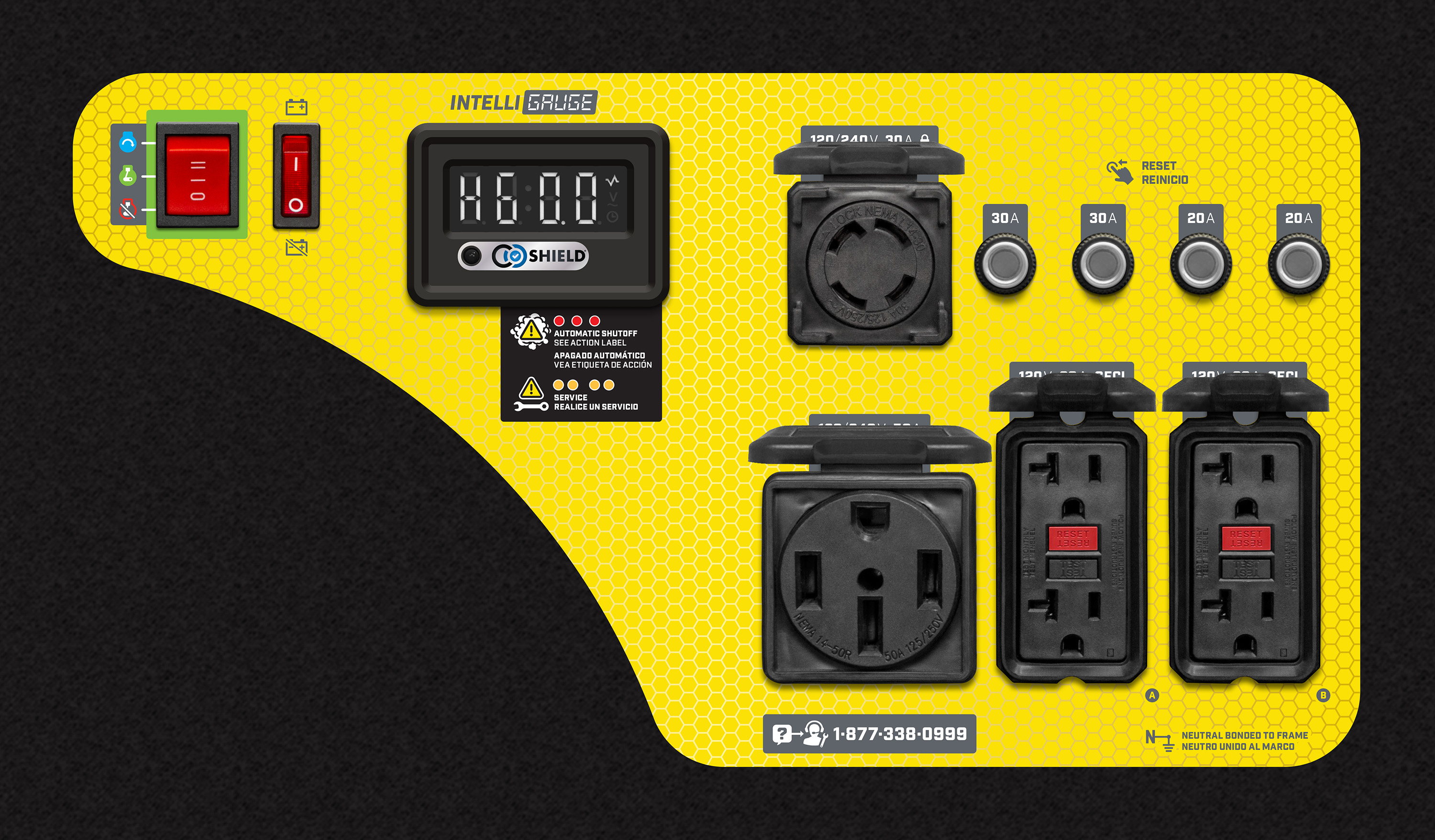8000-Watt Generators

Model: 201035, 201062, 201074
Cycles through voltage, frequency (hertz), run time, and total run time.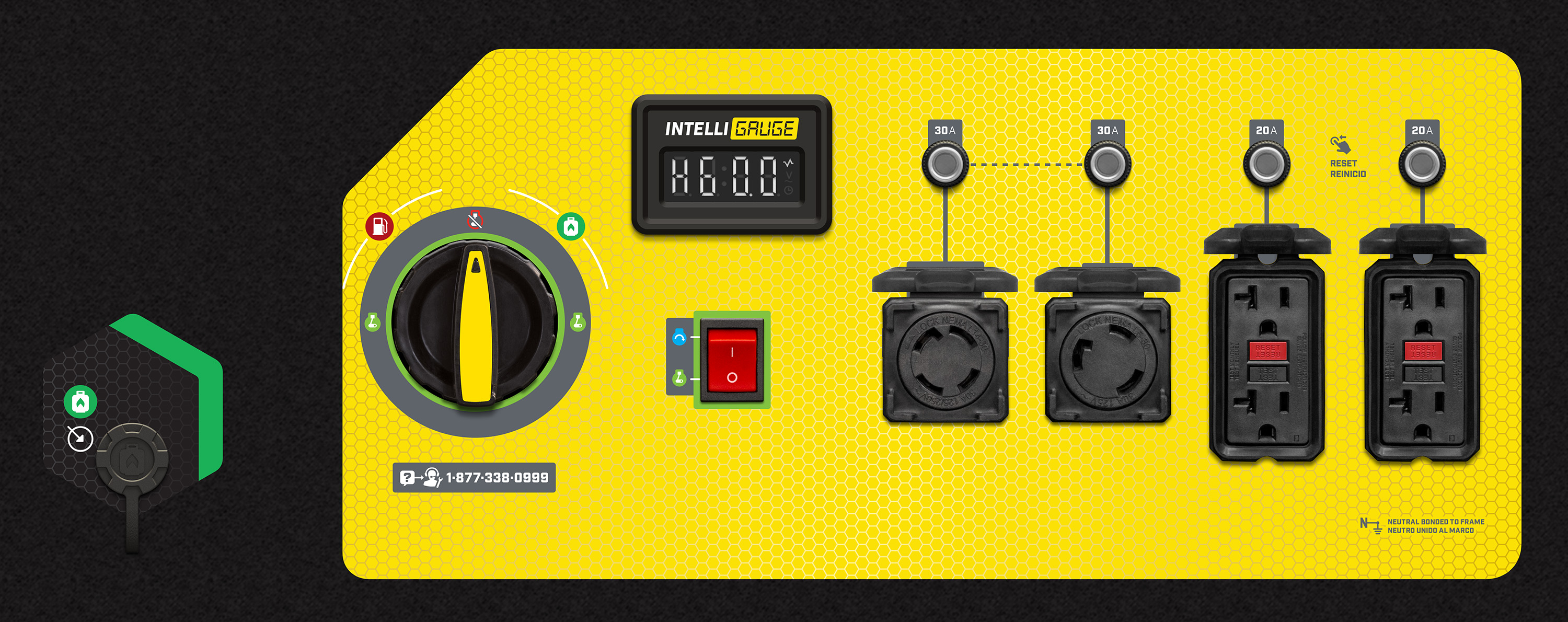Model: 100297
Cycles through voltage, hertz, and total run time.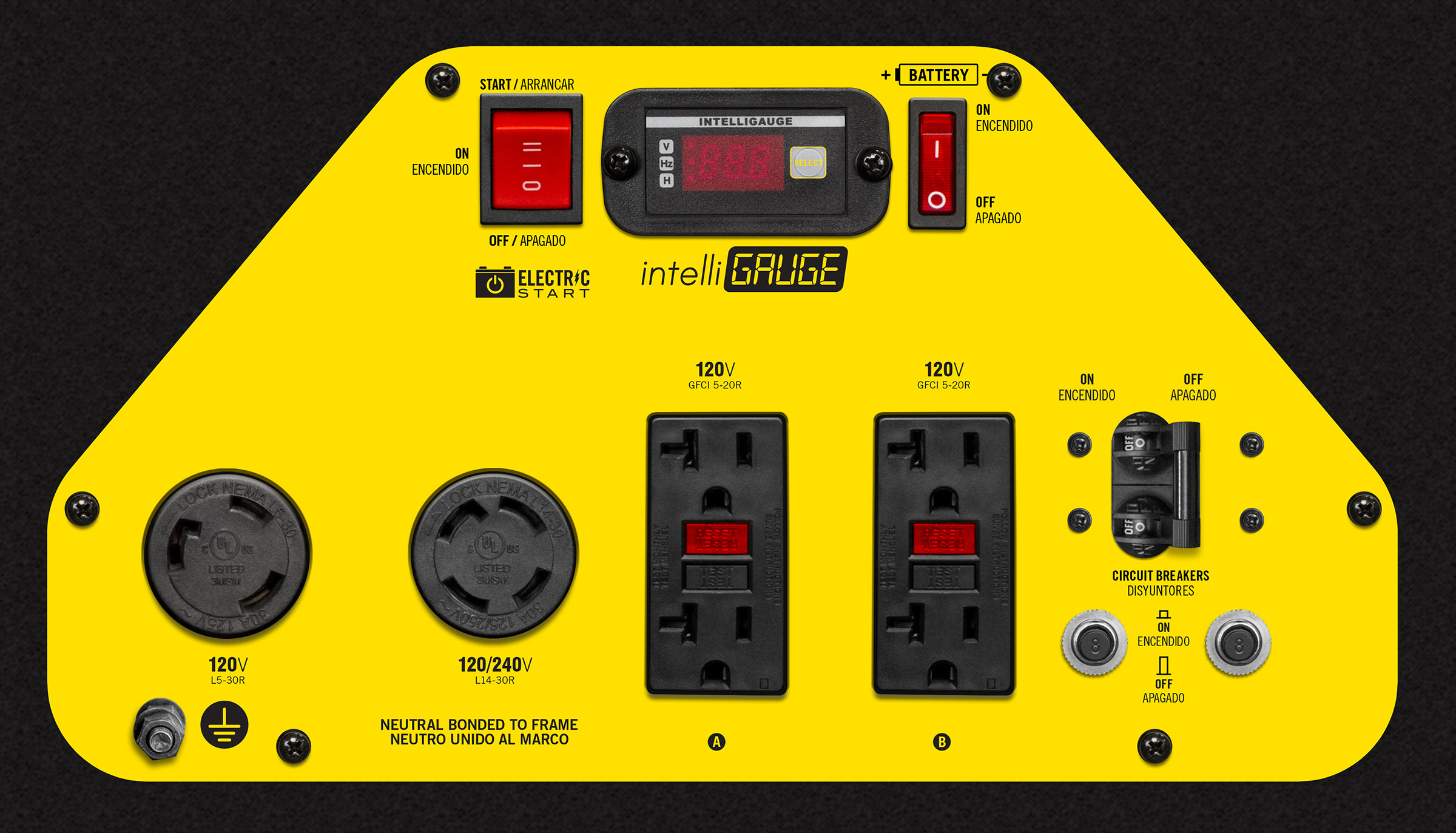Model: 100416, 201078
Cycles through voltage, frequency, run time, and total run time.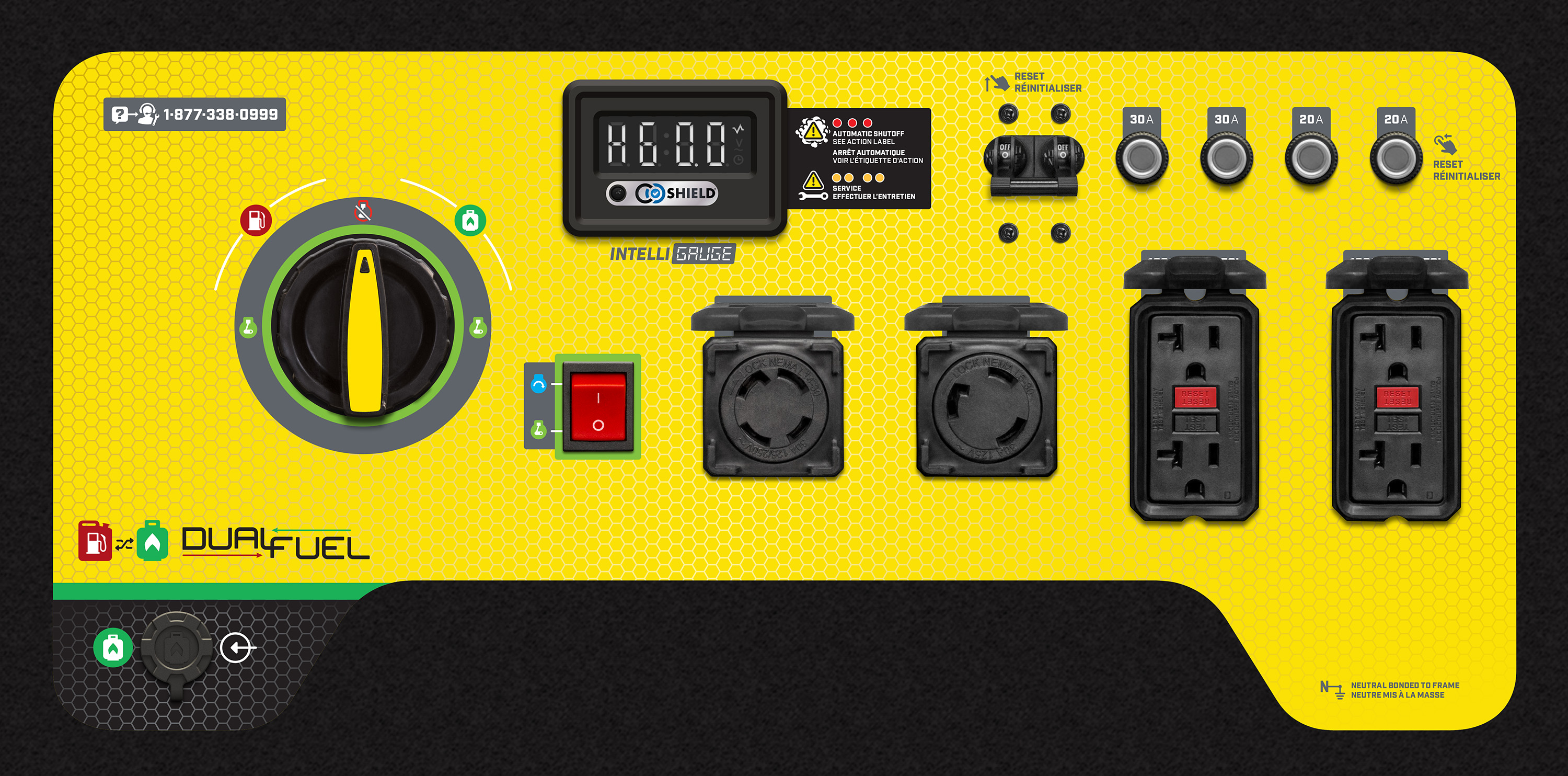8500-Watt Generators

Model: 201083
Cycles through voltage, frequency, session run time, total run time.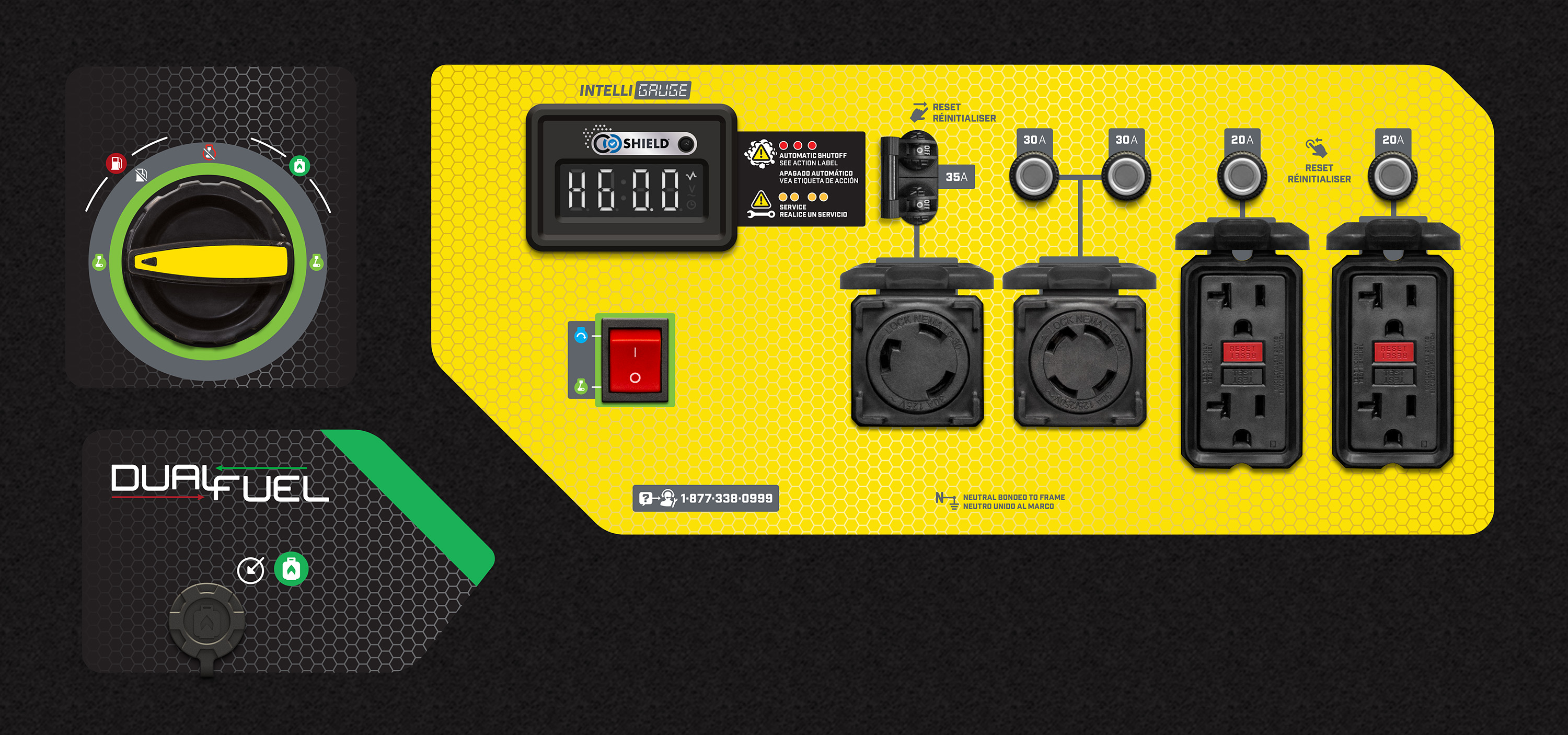8750-Watt Inverters

Model: 100520
Cycles through voltage, frequency (hertz), run time, and total run time.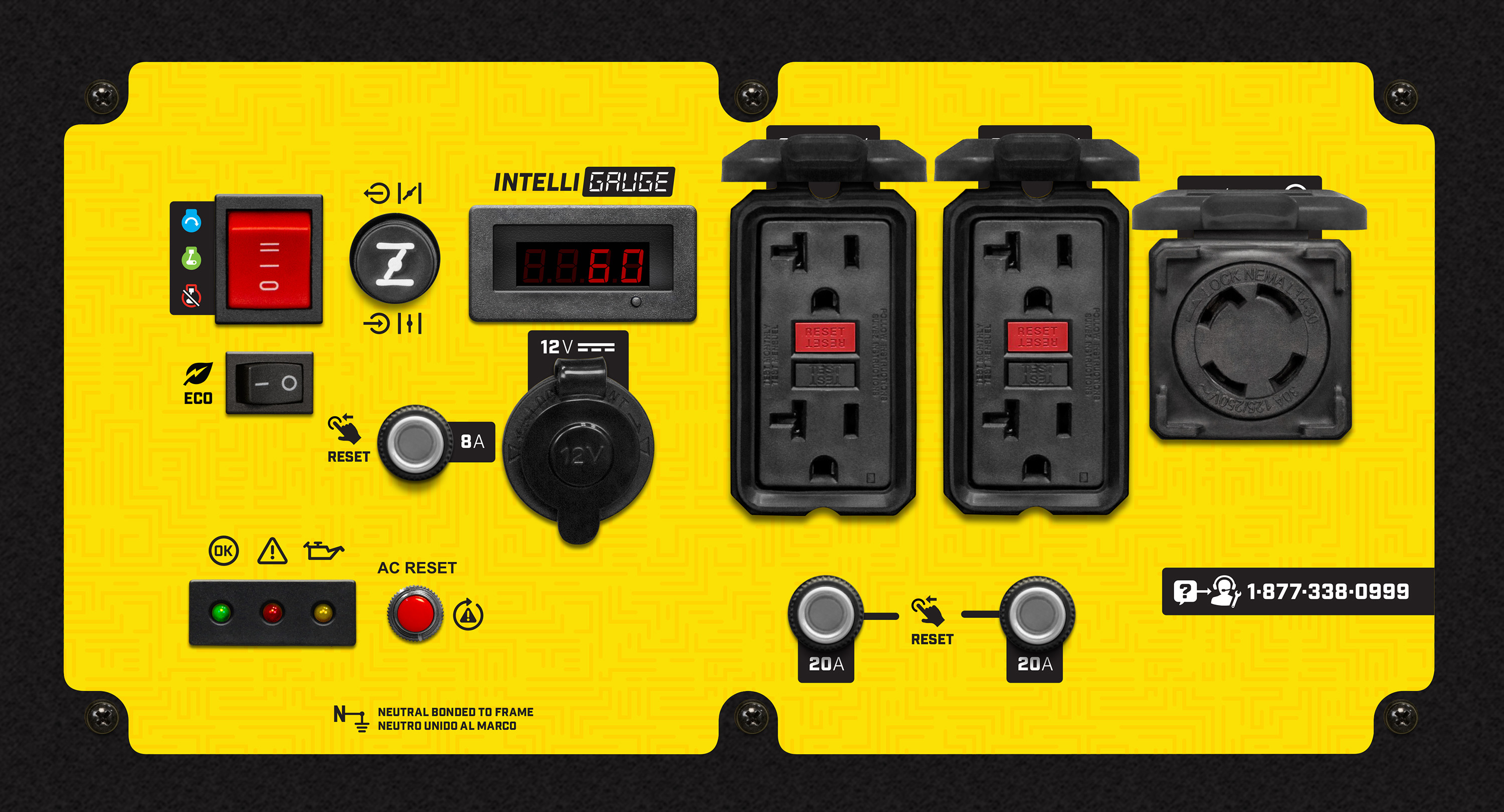9000-Watt Generators

Model: 100637
Cycles through run time, total time, voltage, hertz and power output.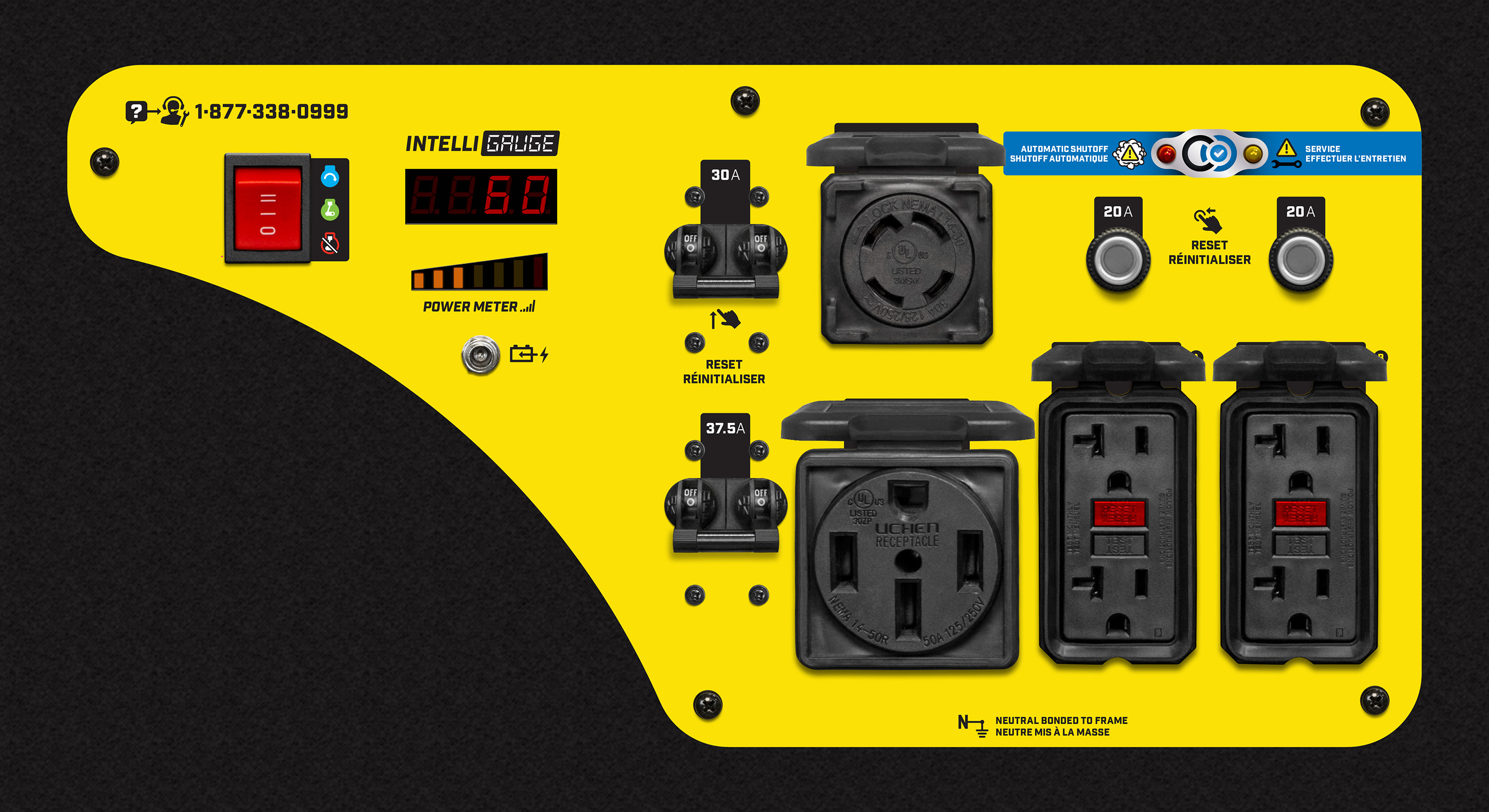9000-Watt Open Frame Inverters

Model: 201067
Cycles through volts, frequency, run time, total run time, and power meter.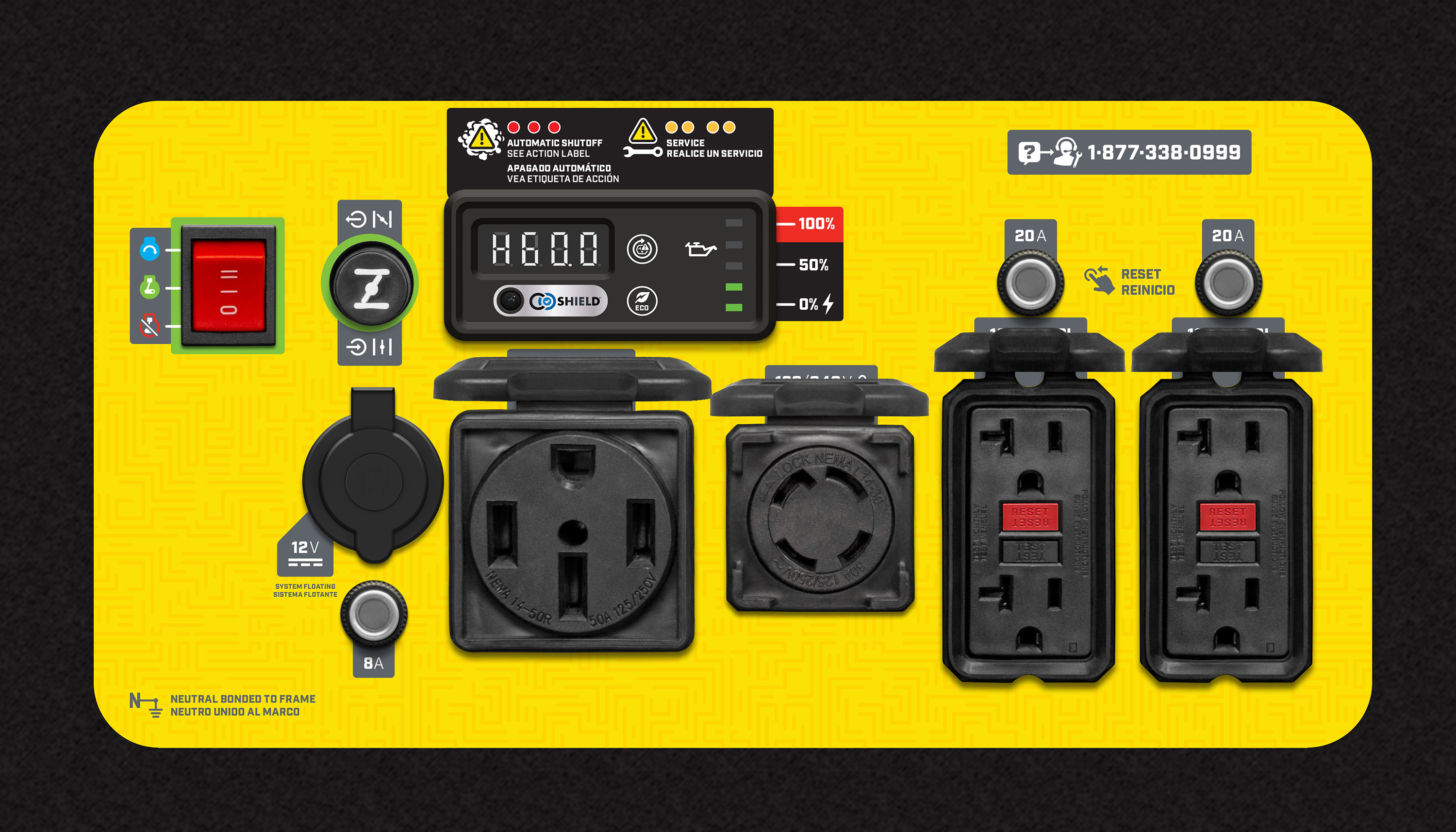9200-Watt Generators

Model: 100110, 100485
Cycles through running hours, total time, voltage, hertz, and wattage output.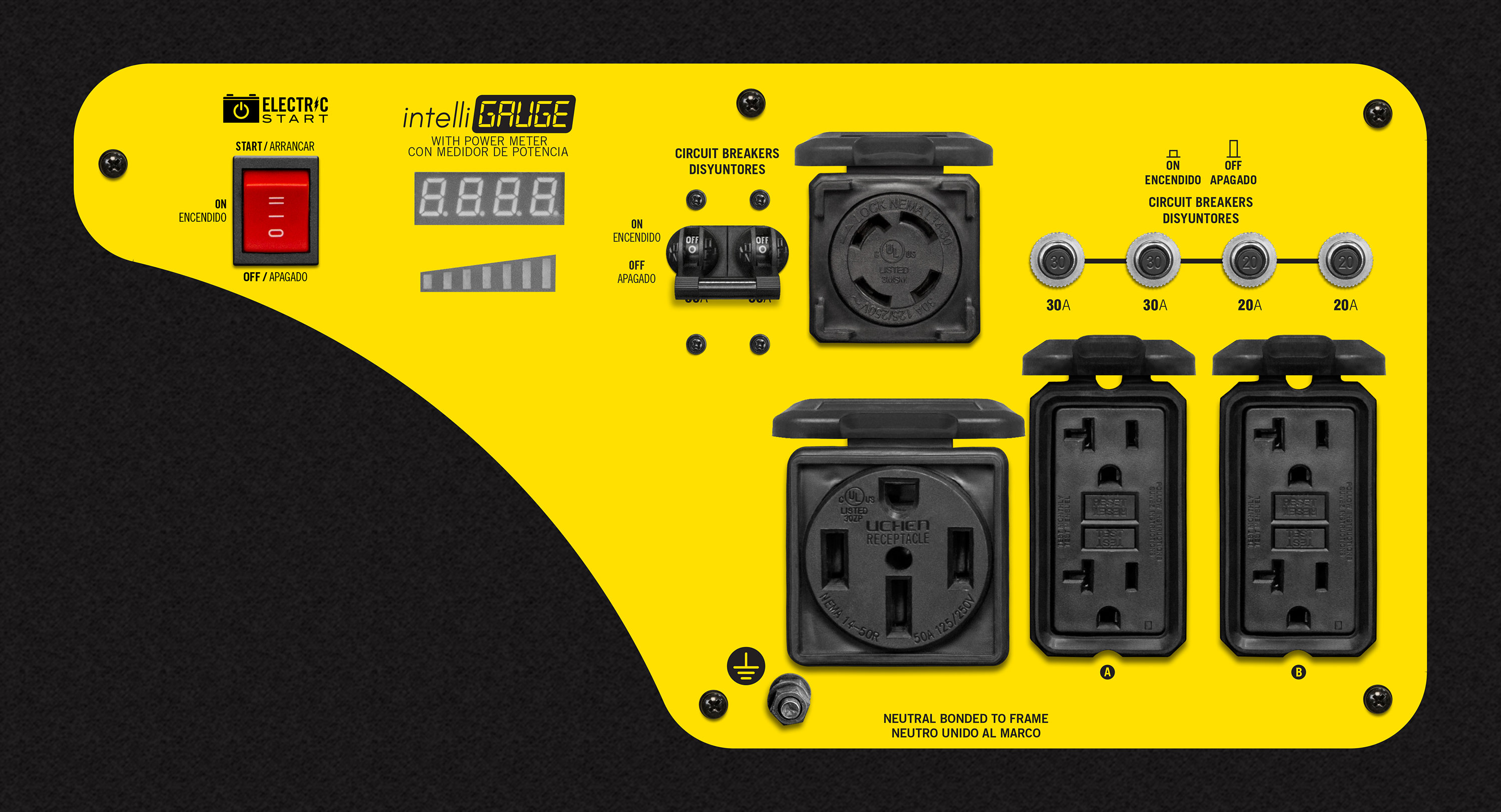Model: 201110
Cycles through voltage, frequency, run time, and total run time.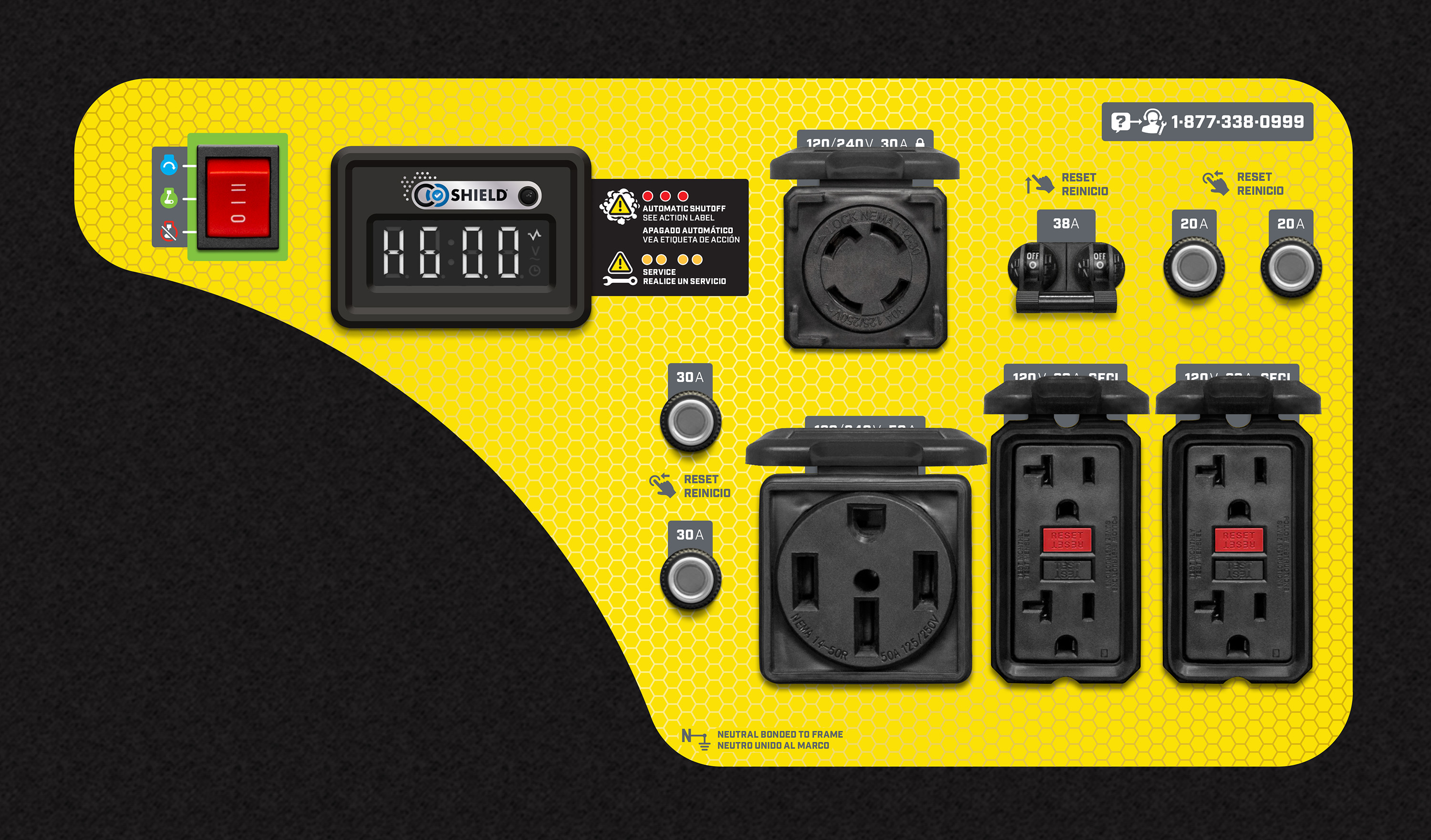9500-Watt Generators

Model: 201043
Cycles through voltage, frequency, run time, and total run time.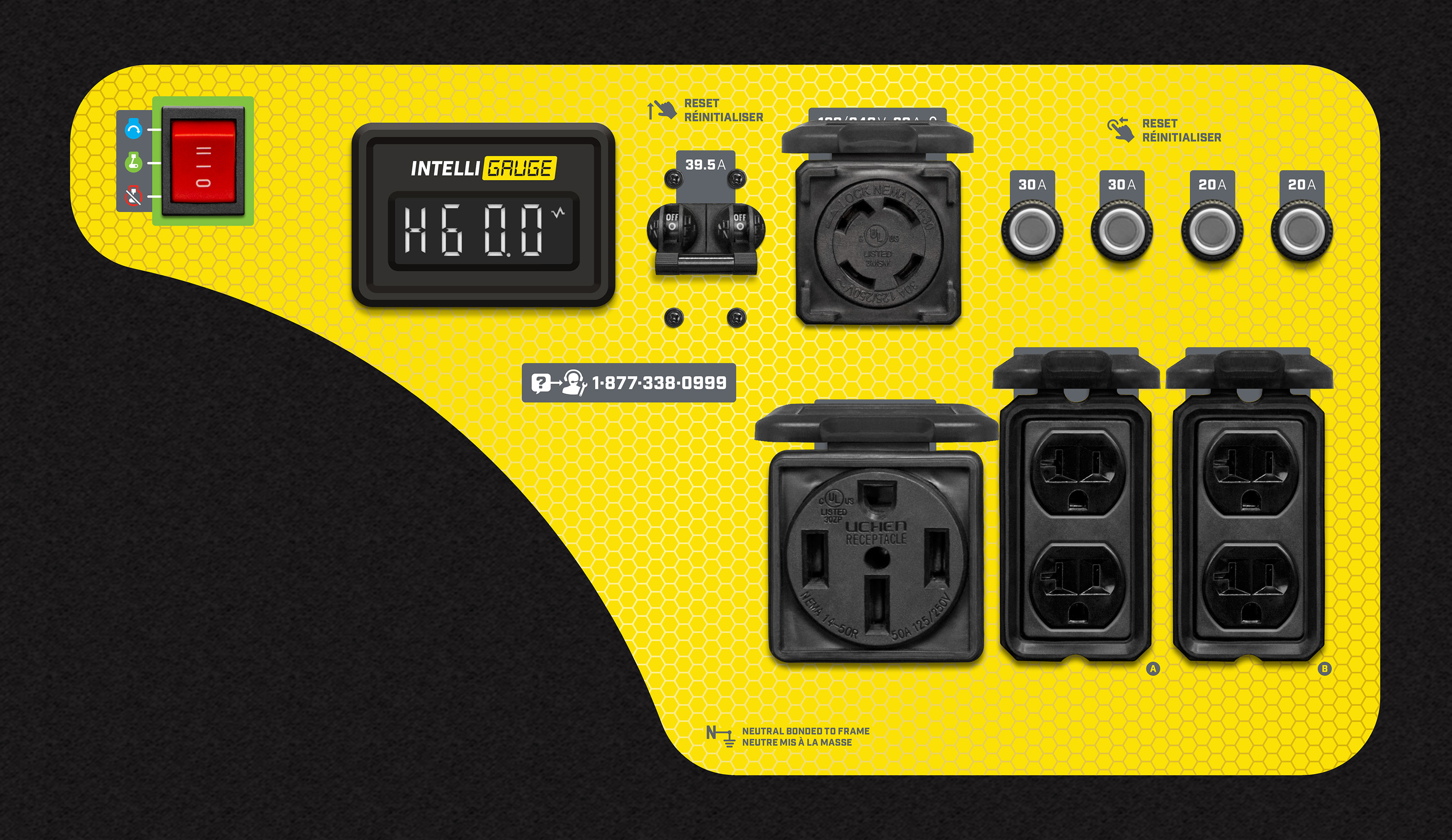Model: 200929
Cycles through voltage, frequency, run time, and total run time.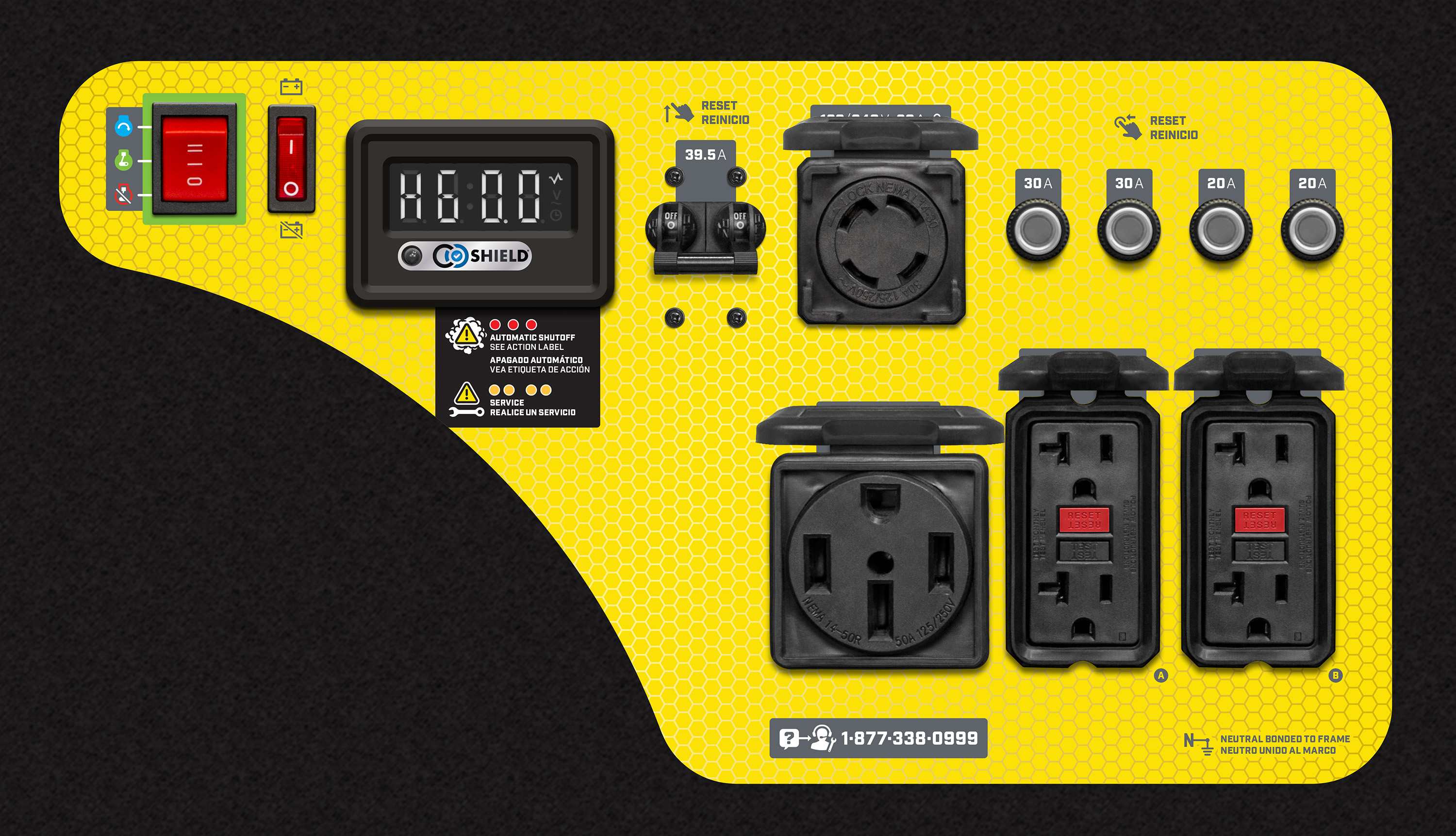10,000-Watt Generators

Model: 201075
Cycles through run time, total time, voltage, hertz and power output.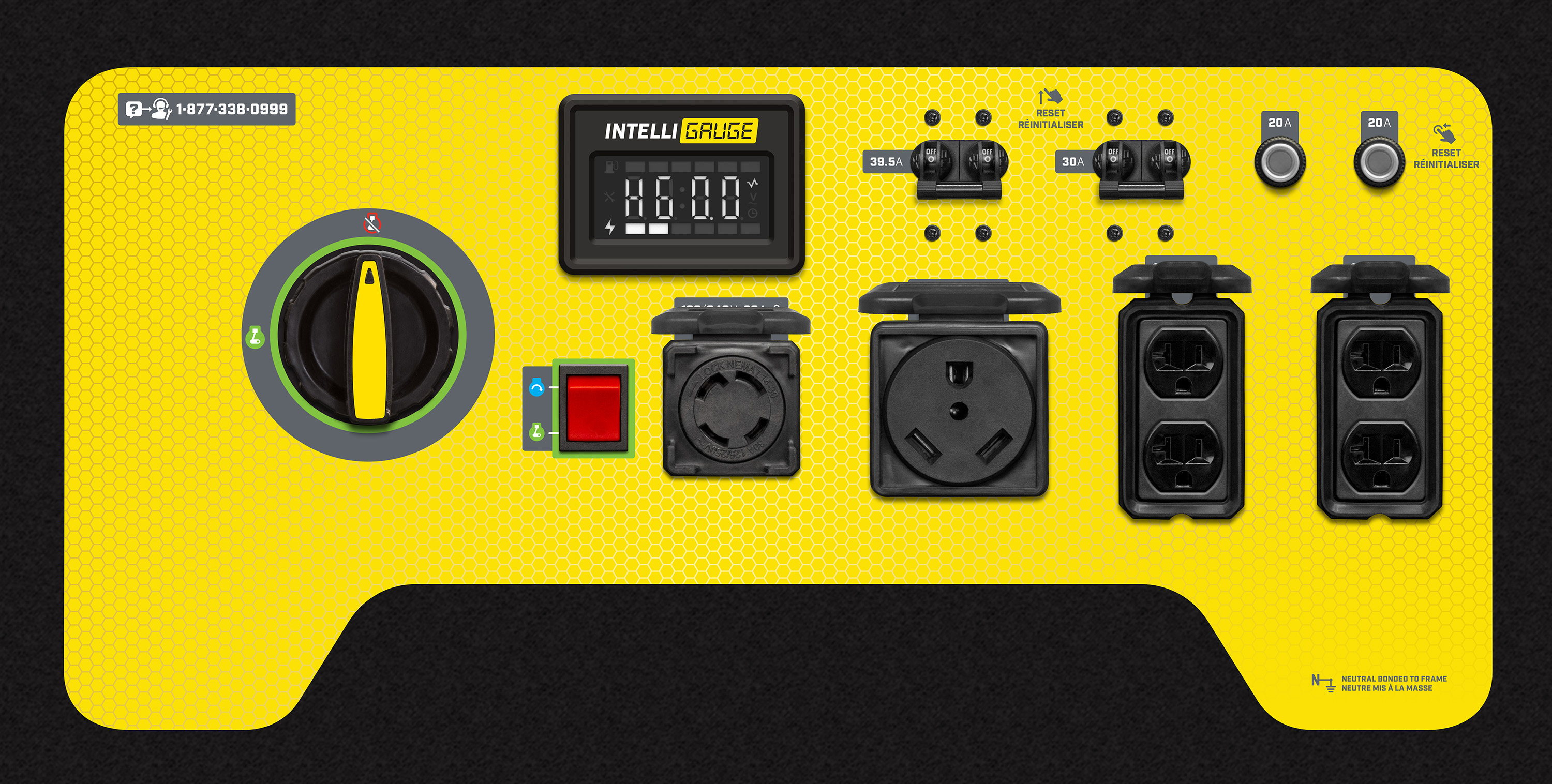12,000-Watt Generators

Model: 100111
Cycles through run time, total time, voltage, hertz, and power output.